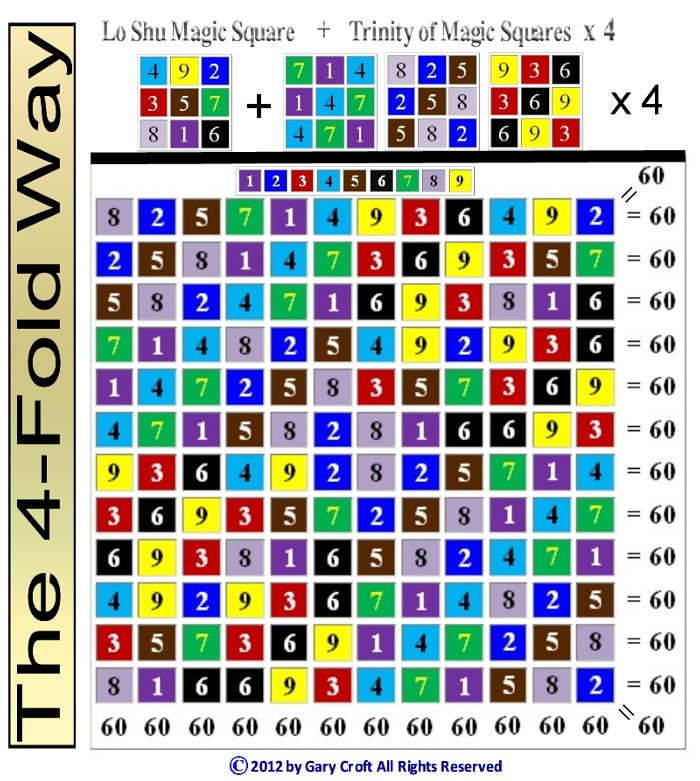# Twin Primes Demystified

### Twin Prime Distribution Algorithms and Symmetries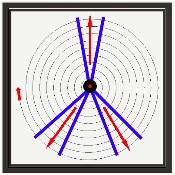This work is licensed by its author, Gary William Croft, under a

"This sieve is the source of two efficient prime number factorization algorithms and completely deconstructs the twin prime sequence." – Philip Gervasse Jackson, citing the Prime Spiral Sieve in Simplicity Instinct: Why Prime Numbers are Elusive!

## Twin Primes: Hidden Dimensions and Symmetries

The factorization algorithms in tandem with rotating symmetry groups that ultimately determine the distribution of twin primes (p, p+2 or 'prime numbers with a gap of two') are fascinating to behold. Below we demonstrate how the Prime Spiral Sieve generates these symmetries in the form of algorithmically permutated mapping matrices, palindromes, rotating equilateral triangles, and complex polygons. The latter include {9/3} star polygons and the beautiful 'palindromagon,' the construction of which sequences the six possible permutations of {3,6,9} palindromically; namely:

639→693→963←369←396←936

[Note: If you're thinking this is 'sacred math,' you'd be mistaken. While it's true that these symmetries are transcendently beautiful, they are rooted in elementary modular and digit sum arithmetic augmented with the most basic geometry. Numinousness is in the eye of the beholder.]

### Twin Prime Distribution Channels

We begin our exploration of twin primes by spiraling numbers congruent to {1, 7, 11, 13, 17, 19, 23, 29} modulo 30 (otherwise defined as natural numbers not divisible by 2, 3 or 5) within a modulo 30 factorization wheel (see graphic, below), where 1 = 12°. By definition this sequence consists of the number one (1) and all prime numbers greater than 5 and their multiplicative multiples, the latter starting with 7 x 7 = 49, the first composite number in the factorization domain. (Ref: http://oeis.org/A007775)

Configuring the spiraling sequence thus reveals that all twin prime candidates (n, n+2), and confirmed twin prime pairs (p, p+2) greater than (5,7) parse along three distinct "distribution channels," i.e., starting with (11,13), potential twin primes are separated by 24° and are parsed equally along three sets of paired radii: {12° ↔ 348°} ... {132° ↔ 156°} ... {204° ↔ 228°} ... in spiraling Archimedean intervals of 30: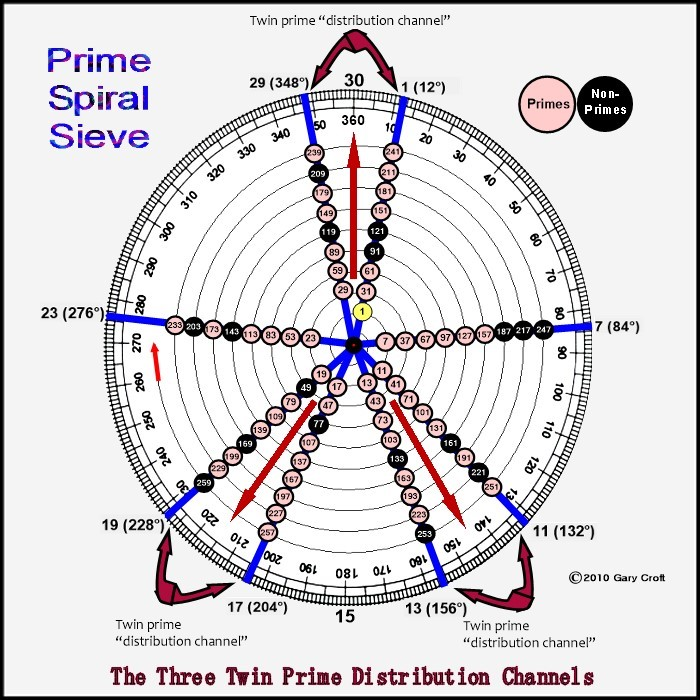Below we translate the three twin prime distribution channels into the language of modular and digit sum arithmetic and express them as functions. To account for composite numbers within our domain, we then explore the beautiful clockworks of modulo 90 factorization by cycling 242 = 576 digital root dyads in period-24 sequences to construct the tiers of 3 x 3 matrices which in turn rotate the vertices of equilateral triangles, i.e., {1,4,7}, {2,5,8}, {3,6,9}, within {9/3} star polygons. Also indicated are the digital roots of the (n, n+2) dyad sums, which – for reasons we demonstrate below – must be either 3, 6 or 9, while the digital root of their n(n+2) products must be 8 in all three cases.

[Note: On this site digital root (also known as repeated digit sum, iterated sum-of-digits, or modulus 9 function) refers to the single digit (1-9) that results when all digit sums are summed in turn, e.g., 47 = 4+7 = 11 = 1+1 = 2; and thus the digital root of 47 = 2, or dr(47) = 2. This in turn reflects the fact that any natural number is congruent modulo 9 to its digital root.]

Numbers ≡ {11,13} modulo 30 (at 132° & 156°) sequence as:

(11,13) = digital roots {2,4} → 2 + 4 = digital root 6
(41,43) = digital roots {5,7} → 5 + 7 = digital root 3
(71,73) = digital roots {8,1} → 8 + 1 = digital root 9
{digital roots repeat...}

Numbers ≡ {17,19} modulo 30 (at 204° & 228°) sequence as:

(17,19) = digital roots {8,1} → 8 + 1 = digital root 9
(47,49) = digital roots {2,4} → 2 + 4 = digital root 6
(77,79) = digital roots {5,7} → 5 + 7 = digital root 3
{digital roots repeat...}

Numbers ≡ {29, 1} modulo 30 (at 348° & 12°) sequence as:

(29,31) = digital roots {2,4} → 2 + 4 = digital root 6
(59,61) = digital roots {5,7} → 5 + 7 = digital root 3
(89,91) = digital roots {8,1} → 8 + 1 = digital root 9
{digital roots repeat...}

All of the n, n+2 twin prime candidates listed above sequence their digital roots in one of three variations: {2,4}, {5,7} or {8,1}. We prove why this is so for all twin prime pairs (11,13) or greater in our Proof by Construction for the Digital Root Sequencing of Twin Primes. This Proof simultaneously demonstrates that all twin prime candidates (n, n+2) greater than (5,7) distribute to one of three modulo 30 dyadic sequences, i.e., numbers ≡ to {11,13}, {17,19} or {29,1} modulo 30.

In our 'Foundations' section, we stated that the set of natural numbers not divisible by 2, 3 or 5 can be expressed as n ≡ {1, 7, 11, 13, 17, 19, 23, 29} modulo 30; once factor-sieved leaving all primes > 5, including all twin primes greater than (5,7). Sub-sorting (to use a spreadsheet term) the prime pair candidates in the twin prime distribution channels can be expressed as:

n ≡ {1, 11, 13, 17, 19, 29} modulo 30

1{+10+2+4+2+10+2} {repeat ...}.

This narrows the factor-sieving field from 8/30 to 6/30, or exactly 20% of natural numbers. We've documented this sequence on the On-Line Encyclopedia of Integer Sequences oeis.org Sequence Number A230462.

### Digital Root Sieving, Modulo 90

"It was through my search for meaning that I discovered how important the digital root space is, which in return rewarded me with a look at how elegant and beautiful the world really is." – Talal Ghannam, Ph.D., The Mystery of Numbers Revealed Through Their Digital Root

Framing our factorization domain (natural numbers not divisible by 2, 3, or 5) in modulo 90 rather than modulo 30 terms expands the number of twin prime distribution channels from 3 to 9, as pictured here: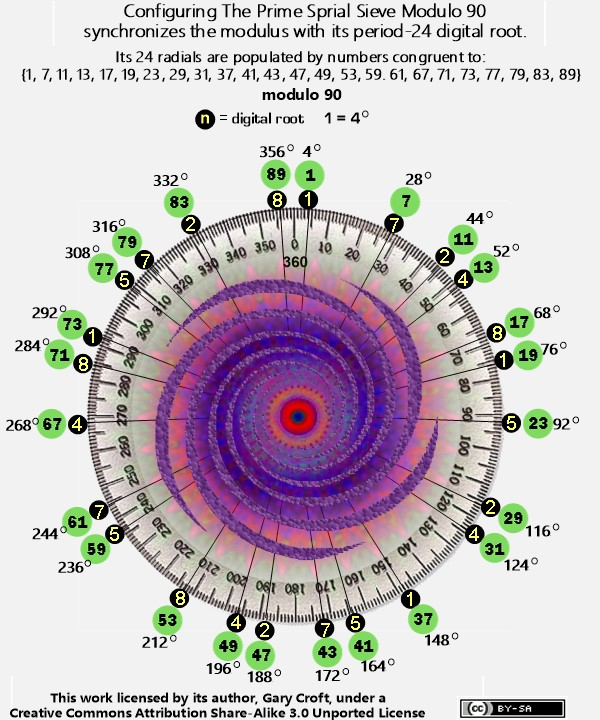The advantage of telescoping our frame of reference to modulo 90 is that it synchronizes the modulus with its period-24 digital root and thus parses twin prime candidates by their digital root dyads {2,4}, {5,7} and {8,1}, as follows:

digital root {2,4} = n ≡ { (11,13), (29,31), (47,49) } mod 90: 11{+2+16+2+16+2+52} {repeat ...}
digital root {5,7} = n ≡ { (41,43), (59,61), (77,79) } mod 90: 41{+2+16+2+16+2+52} {repeat ...}
digital root {8,1} = n ≡ { (17,19), (71,73), (89, 1) } mod 90: 17{+2+52+2+16+2+16} {repeat ...}

Further disaggregating the sieving action, it follows that for all twin primes > (5,7):

digital root {2} = n ≡ {11, 29, 47} mod 90: 11{+18+18+54} {repeat ...}
digital root {4} = n ≡ {13, 31, 49} mod 90: 13{+18+18+54} {repeat ...}

digital root {5} = n ≡ {41, 59, 77} mod 90: 41{+18+18+54} {repeat ...}
digital root {7} = n ≡ {43, 61, 79} mod 90: 43{+18+18+54} {repeat ...}

digital root {8} = n ≡ {17, 71, 89} mod 90: 17{+54+18+18} {repeat ...}
digital root {1} = n ≡ {19, 73, 1} mod 90: 19{+54+18+18} {repeat ...}

Sorting the digital root congruence sets sequentially reveals perfect diagonal and central-vertical 90-sum symmetry (color-coded in the graphic below):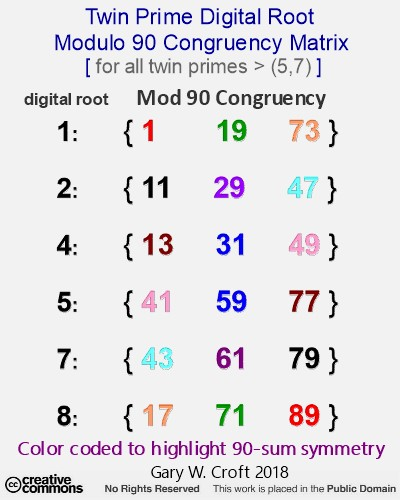The matrix below makes these congruence relationships clear: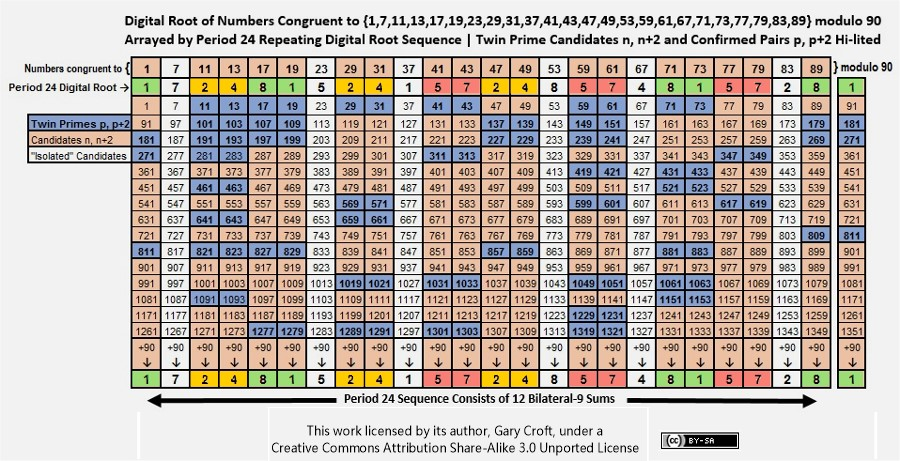This matrix also reveals that the digital root sequencing of the twin prime candidate dyads (starting with 11, 13) is period-18:

{2 4, 8 1, 2 4, 5 7, 2 4, 5 7, 8 1, 5 7, 8 1} {repeat ...}.

We've documented the three twin prime digital root dyad sequences on the On-Line Encyclopedia of Integer Sequences (and we explore the beautiful symmetries they create under the heading "Digital Root Parsing of the Twin Primes," below). Here are the OEIS sequence numbers and links:

### The Trinity of Rotating Equilateral Triangles

Earlier we drew your attention to the fact that the sums of the twin prime digital root dyads must equal 3, 6 or 9. We did so because these represent a triad, {3,6,9}, which – with {1,4,7} and {2,5,8} – are the vertices of rotating equilateral triangles that taken together constitute the "Trinity of Triangles" (illustrated below).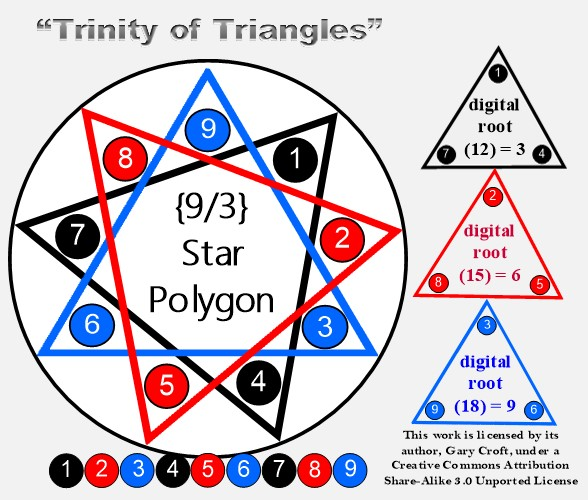Below we will demonstrate how factorization algorithms and twin prime dyad cycling at the digital root level rotate the vertices of equilateral triangles within {9/3} star polygons like the one pictured above. These rotations are encoded in 3 x 3 matrices generated by period-24 digital root dyad tri-level cycling. We will also reveal the 'Latin Square' reflecting {3,6,9} hidden in plain sight betwixt and between the twin prime distribution channels; all of its rows, columns and principal diagonals summing to 18.

### Consolidation of the Twin Prime Distribution Channels

Consolidating the 6 radii used to construct the three twin prime distribution channels into a single number line completely isolates twin prime candidates, pairing them in tandem in a deterministic sequence, starting with (11, 13), thus:

1 {+10+2+4+2+10+2} {repeat ... ∞}.

Noting that the first term in the sequence is 1 + 10 = 11, we find, for example, that six of the first seven such pairings are twin primes (and we should add that all twin primes within this range are accounted for):

(11, 13) (17, 19) (29, 31) (41, 43) (47, 49 = 7x7) (59, 61) (71, 73) ...

(Excluded by definition are numbers ≡ {7, 23} mod 30, sequencing as 7 {+16+14} {repeat ... ∞}. With the exception of 7, members of this sequence cannot form twin prime pairs given their closest possible proximity to neighboring prime numbers is +4 and -4, respectively.)

Below is a matrix showing the distribution of twin prime sets (both candidates and confirmed prime pairs) from (11, 13) to (569, 571):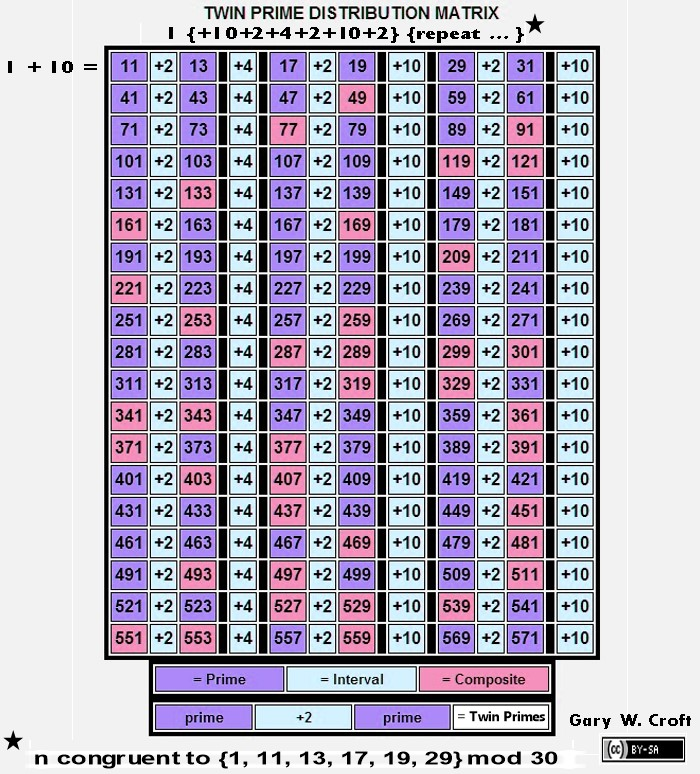The first three twin prime sets in this matrix configuration are an anagram, in angular terms (in a 30-sectioned circle such as encompasses the Prime Spiral Sieve, where 1 = 12°), to the first three primes, thus: (11, 13) (17, 19) and (29, 31) respectively translate to:

11 + 13 = 24 ... 24° = 2
17 + 19 = 36 ... 36° = 3
29 + 31 = 60 ... 60° = 5

[You'll note that the sum of the intervals for each row of twin prime candidates = 2 x 3 x 5 = 30, and that the intervals separating the first 36 twin prime pair candidates sum to 360, i.e., between (11, 13) and (359, 361), bringing the candidate sets full circle, so to speak; and interestingly the product of the sums of the first three twin prime candidate sets = 24 x 36 x 60 = 51840, while 51840/360 = 144 and 51840/432 = 120.]

### Factorization Algorithms for the Twin Prime Candidates

All composite numbers in the twin prime distribution channels can be accounted for algorithmically by the eight-fold modulo 30 progressions shown below where we factor-sieve by division then multiplication.

[Note: The algorithms shown here are a sub-set of the chordal progressions discussed at some length earlier that factored all composite numbers in the array populated by natural numbers not divisible by 2, 3 or 5, as opposed to only factoring those impacting twin prime candidates, demonstrated below.]

The matrix that follows shows factorization by sequential division specific to the twin prime distribution channels. The left-most column consists of the number 1 (which we've dubbed 'silent') and members of the twin prime distribution channels in sequence, thus delimiting the prospective factorization to only twin prime candidates.

Essentially, we've sequentially divided numbers ≡ {1, 11, 13, 17, 19, 29} mod 30 by numbers ≡ {1, 7, 11, 13, 17, 19, 23, 29} mod 30. All modulo n ≡ 0 (i.e., zero remainder) results generated (except when a number is divided by itself) indicate factors (arrayed horizontally, hi-lited in pink) of composite numbers n (arrayed vertically, also hi-lited in pink), while twin primes are hi-lited in bright blue and primes not belonging to a twin prime pair are hi-lited in yellow.

When the square root of any number in the twin prime distribution sequence is reached without producing a remainder of zero, the number must by definition be prime. For example, the square root of 311 is 17.6352... When 311 is divided in turn by 7, 11, 13, and 17 no zero remainders are produced (and, regardless, for obvious reasons 17 can't possibly be one of 311's factors). The next possible factorization, 192 = 361, is out of range, and therefore 311 must be prime. Here's the division factorization algorithm matrix: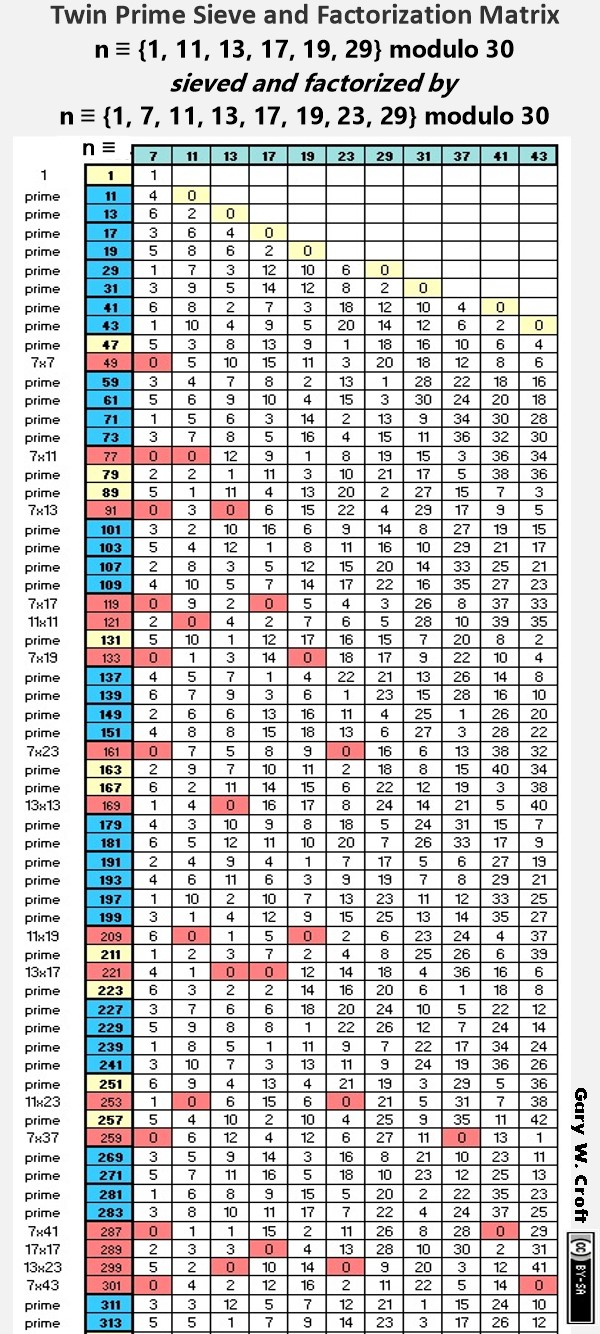Below we show only the calculations required to determine primality of twin prime candidates within the designated range of 11 to 313. This illustrates the significant increase in sieving efficiency that results from the fact that you need only activate divisors when their squares are reached. For example, you needn't begin dividing by 11 until its square, 121, is reached. And of course, from a programmer's perspective, the sieve has another built-in efficiency in that division into a given twin prime candidate stops immediately upon encountering a remainder of zero (0) identifying it as composite: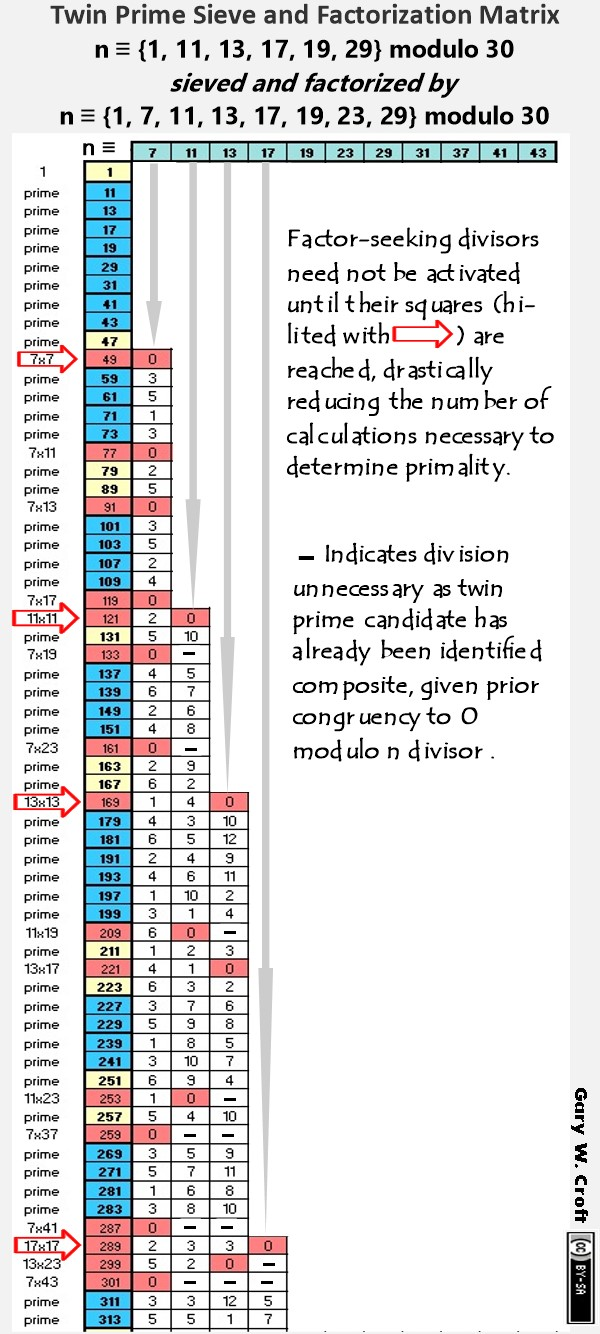Multiplicative factorization sequencing (illustrated in the graphic below) is simply a reversal of the division process shown above. This algorithm is self-evidently more efficient than it's division sibling given every 'beat' indexes (identifies) a composite number (Although we should caution, as we detail under Counting Primes, some composite numbers get 'hit' more than once. These redundant factorizations do put a measure of drag on efficiency.). Given that in this case we're illustrating the sequencing in a modulo 90 frame, those factorizations impacting twin primes are n ≡ {1, 11, 13, 17, 19, 29, 31, 41, 43, 47, 49, 59, 61, 71, 73, 77, 79, 89} modulo 90 (initiated by 7 * 7= 49, as 1 is 'silent'). From this, it shouldn't be surprising to learn that the density of prime numbers as a percentage of real numbers graphs roughly at a 45° angle–a mirror image of the factorization geometry, which we can think of as a graphic representation of factorization density.### Twin Prime Distribution Channel Functions

The three twin prime distribution channels can be defined as functions, where:

x = natural numbers 1, 2, 3, ...
f(x) = 30x -19, -17 ...
f(x) = 30x -13, -11 ...
f(x) = 30x -1, +1 ...

Below we delineate the functions with examples (the first two twin prime candidate dyads generated by each):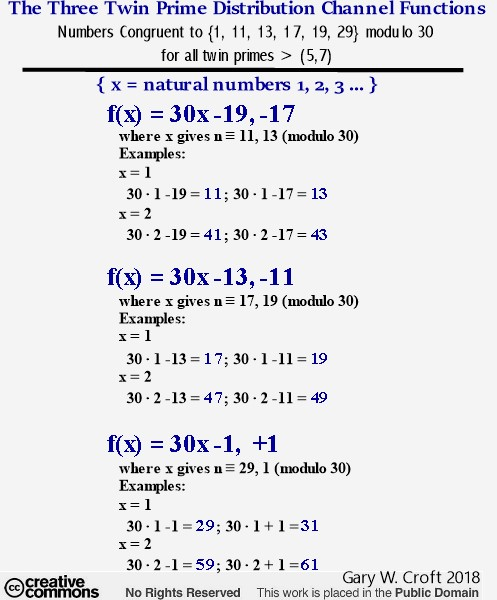### Digital Root Multiplication Dyads: The 36 Fundamental Operations of Modulo 30 Factorization

"Vedic Mathematics is...a sophisticated pedagogic and research tool ... ." – Dr. L.M. Singhvi, former UK High Commisioner for India, from his forward to Cosmic Calculator, by Williams & Gaskell

Analyzing the factorization algorithms discussed thus far from a digital root perspective leads us to the 6 x 6 = 36 fundamental operations of modulo 30 factorization. Below we illustrate a Vedic Square (an ancient digital root multiplication matrix attributed to Indian mathematicians) being 'lightened' by removing its 3's, 6's and 9's. This was done to reflect the fact that the digital roots of our modulo 30 factorization domain are restricted to 1, 2, 4, 5, 7, and 8 (and we note that technically the subset {1, 2, 4, 5, 7, 8} forms a cyclic group of multiplicative units in the ring Z/9Z, forming the 6 x 6 = 36-cell Latin Square below we've dubbed 'Vedic Square Light'):Reconfiguring our 'Vedic Square Light' to parse the factor dyad products by digital root gives us the 36 fundamental digital root factorization dyads: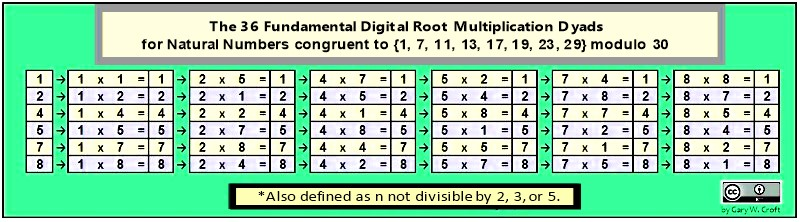Can you find this 36-digit palindrome weaving through the matrix above like two opposing square waves?:
1, 2, 4, 5, 7, 8, 4, 8, 7, 2, 1, 5, 7, 5, 1, 8, 4, 2, 2, 4, 8, 1, 5, 7, 5, 1, 2, 7, 8, 4, 8, 7, 5, 4, 2, 1

Like a coin sorter, below we parse the first 41 elements of our domain as they naturally sequence under digital root dyads {2,4} ... {8,1} ... {5,7} (albeit with algorithmically determinable gaps). This range starts with the number 1, and includes 33 prime numbers (10 pairs of which form twin primes) and 7 composite numbers (for which we display both natural and digital root factors):### Modulo 90 Congruence Matrix

The matrices below reveal that the factorization algorithms generated within our domain possess perfect symmetry when the products are converted to their modulo 90 congruence and framed by their period-24 horizon. For example, 7 x 17 = 119 which is congruent to 29 (modulo 90). On the matrix below you will find that n ≡ 7 mod 90 x n ≡ 17 mod 90 = n ≡ 29 mod 90. And note that the additive sum of all columns and rows = 1080 = 360 x 3, while every row and column consists of 12 bilateral-90 sums and 12 bilateral-10 terminating digit sums, and all diagonals are palindromic. Here's the matrix:Two of the palindromic diagonals in the above matrix demand special attention: For one, the period-24 principal diagonal (upper-left to lower right hand corners, color-coded blue) captures the modulo 90 congruence of all the perfect squares in our factorization domain. In modulo 90 terms they are:

n ≡ {1, 49, 31, 79, 19, 1, 79, 31, 61, 19, 61, 49, 49, 61, 19, 61, 31, 79, 1, 19, 79, 31, 49, 1} mod 90

... which reduces to:

n ≡ {1, 19, 31, 49, 61, 79} modulo 90

... which in turn is a subset of the twin prime candidate distribution sequence for all twin primes > (5,7):

n ≡ {1, 13, 17, 19, 29, 31, 41, 43, 47, 49, 59, 61, 71, 73, 77, 79, 89} modulo 90.

This construction thus forms the basis for proving that the squares of all natural numbers not divisible by 2, 3 or 5 must have digital root of either 1, 4, or 7 and that they negate twin prime candidacy, the first 'casualty' being (47 [prime], 49 = 72).

The other palindromic sequence of special interest is the secondary diagonal (lower-left to upper-right hand corner; color-coded red). It consists exclusively of prime numbers and is point-counterpoint to the sequence of modulo 90 squares, detailed above:

n ≡ {89, 41, 59, 11, 71, 89, 11, 59, 29, 71, 29, 41, 41, 29, 71, 29, 59, 11, 89, 71, 11, 59, 41, 89} mod 90 {repeat …}.

It reduces to prime numbers {11, 29, 41, 59, 71, 89} x 4 = 1200 with digital roots restricted to 2, 5, and 8 (and note 11+89=100; 29+71=100; 41+59=100).

The sequence can also be derived formulaically from the squares of n ≡ {1, 7, 11, 13, 17, 19, 23, 29} mod 30, noted above (framed mod 90; period-24). Here’s the formula:

a(n) = 90 − ( n ≡ {1, 7, 11, 13, 17, 19, 23, 29}(n)2 mod 90).

The first 9 terms in this sequence compute to:

For n = 1: k = 90 − mod 90 of ^2 gives k = 89;
For n = 7: k = 90 − mod 90 of 2 gives k = 41;
For n = 11: k = 90 − mod 90 of 2 gives k = 59;
For n = 13: k = 90 − mod 90 of 2 gives k = 11;
For n = 17: k = 90 − mod 90 of 2 gives k = 71;
For n = 19: k = 90 − mod 90 of 2 gives k = 89;
For n = 23: k = 90 − mod 90 of 2 gives k = 11;
For n = 29: k = 90 − mod 90 of 2 gives k = 59;
For n = 31: k = 90 − mod 90 of 2 gives k = 29...

Summing the principal and secondary diagonals gives us 1200 + 960 = 2160 = 360 * 6 = 432 * 5. And aligning the principal and secondary diagonals forms this string of 24 dyads summing to 90 each, again for a total of 2160 (and note that only terminating digits 1 and 9 are present and that there are also 24 diagonal dyads summing to 90 each):Next, we provide two examples of the 24 sets of 24 multiplicative dyads, highlighting the products of the 24 dyads that produce results congruent to {1 and 71} modulo 90, followed by lists of the dyads themselves. And we note that the sets of 24 each n congruent to {1 and 71} modulo 90 dyads display perfect end-to-end bilateral 90-sum symmetry which translates to perfect 9-sum symmetry at the digital root level (and this holds true for all 24 sets). [Here's a link to an Excel spreadsheet that documents all 24 sets of 24 factorization dyads. And they are presented in tables further down this page, as well.]

Study these, and you'll come to the profound realization that the 24 sets can be visualized end-to-end as a spiral with 288 counter-balancing 90-sums on the multiplier side and rotational mirroring on the multiplicand side for another 288 = 122 x 2 bilateral 90 sums. This 24-fold concatenation of symmetries at the heart of nature is truly awe inspiring, especially when you consider that they ultimately determine the distribution of all prime numbers > 5 (while the first three primes {2, 3, 5} and their primorial 30 construct the scaffolding within which the algorithmically generated factorization dyads operate):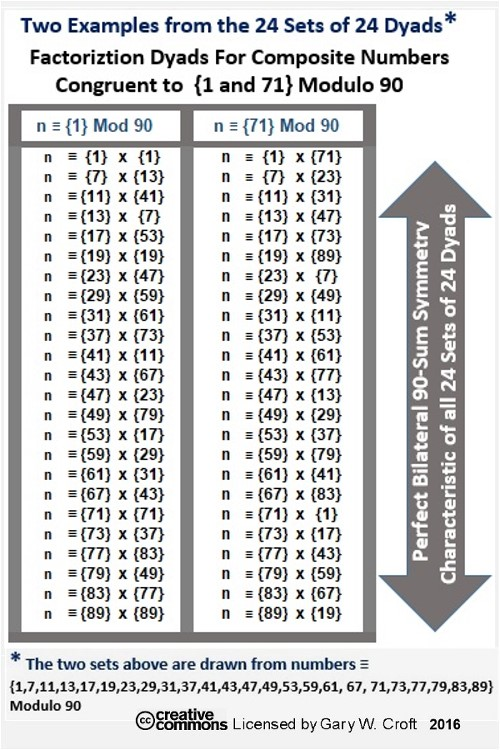[Note: The 24 factorization dyads listed above that account for all composite numbers congruent to {1} modulo 90 give one pause when you consider that there are exactly 1000 prime numbers between 1x1 and 89x89. And we remind you that 89 is the 24th prime number and the 24th natural number not divisible by 2, 3, and 5, and that 242 = 576, the total number of factorization dyads in our modulo 90 defined domain (arrayed in the tables that follow). And we note that 5 * 7 * 6 = 210 = the first 4 primorial primes: 2 * 3 * 5 * 7 and that 12 * 22 * 32 * 42 = 576. Another interesting feature of 576 is that the cumulative digital root for natural numbers 1, 2, 3 ..., i.e., {1 + 2 + 3 + 4 + 5 + 6 + 7 + 8 + 9} {repeat ...}, from 1 to 576 = 2880 = 8 * 360 = 5 * 576; and 576 + its cumulative digital root 2880 = 3456 and 3 * 4 * 5 * 6 = 360. Also, the cumulative digital root of 24 = the 2nd repunit 111 and 111 = (1 * 102) + ( 1 * 10) + (1 * 1). The undersigned is convinced none of this is coincidental.]

The tables below account for all 24 sets of 24 dyads: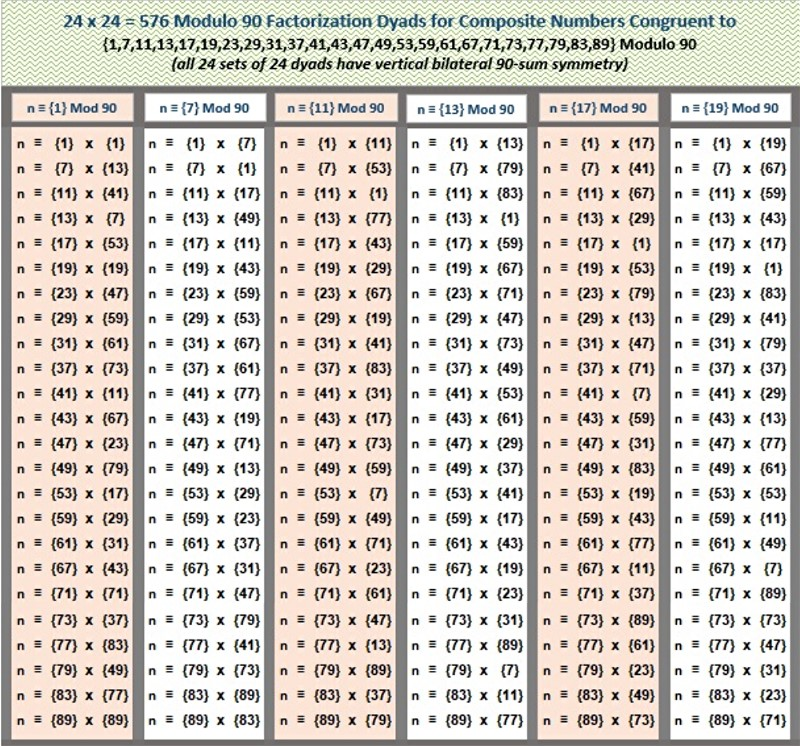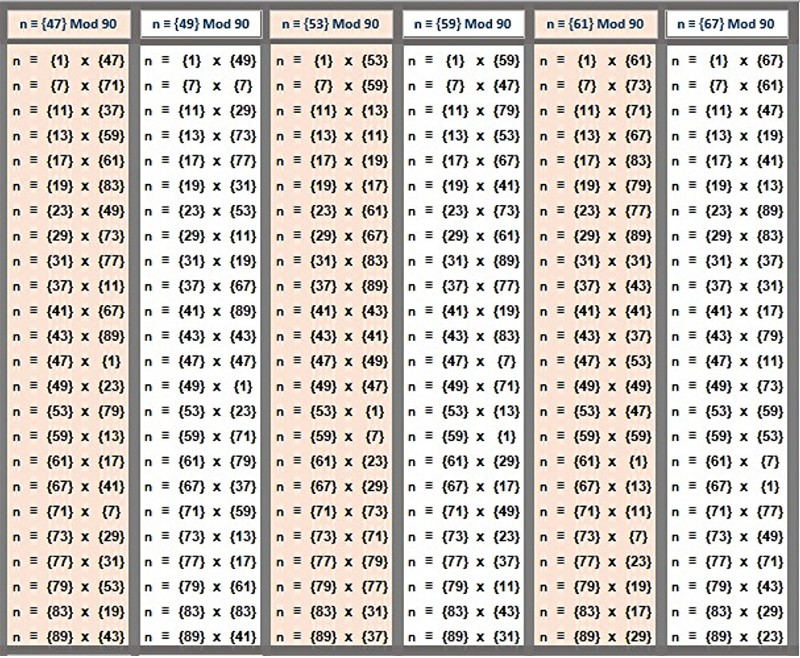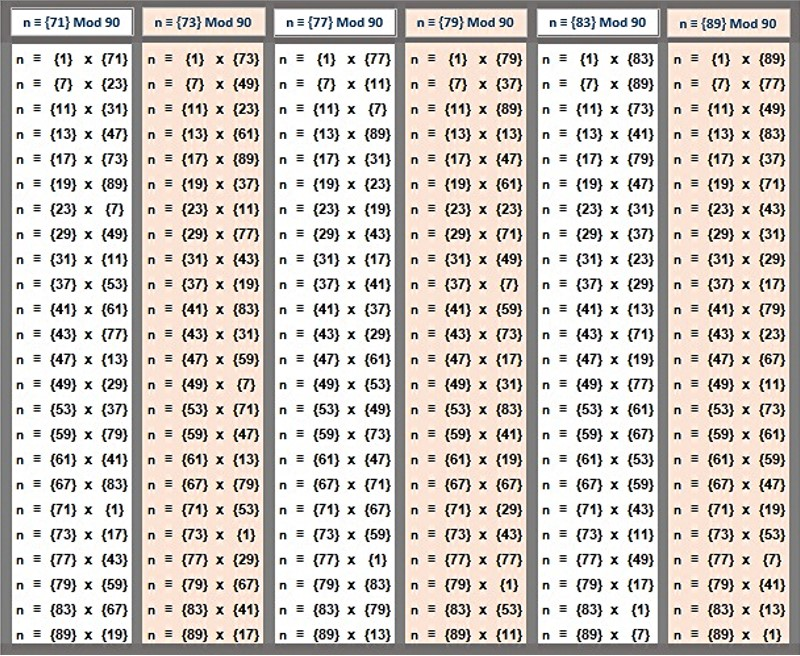Reflecting back on the 6 x 6 = 36 fundamental digital root operations of modulo 30 factorization, below we've organized the 576 modulo 90 factorization dyads into 36 sets of 16 each, parsed by digital root dyads. The result–a factorization clockworks–is profoundly beautiful, especially given these dyads combinatorialy generate period-24 cycles that codify the construction of 576 {9/3} star polygons (discussed and illustrated in detail down this page under the heading, "Period-24 in All Directions.").

We conjecture that these constructions are manifold to what is known as Equilateral Triangle Lattice Universal Optimality (for which there is considerable interest in finding a proof). The 24-dimension algorithmically constructed symmetry groups described here involving the rotating vertices of equilateral triangles within {9/3} star polygons provide both the 24- and 9-dimensional geometries needed for a proof). This is related in turn to Maryna Viazovska's R24 optimal sphere packing theorem, referenced (with a link to her paper) below in the section headed "Seeing Stars: Rotational Symmetry Groups, Modulo 90."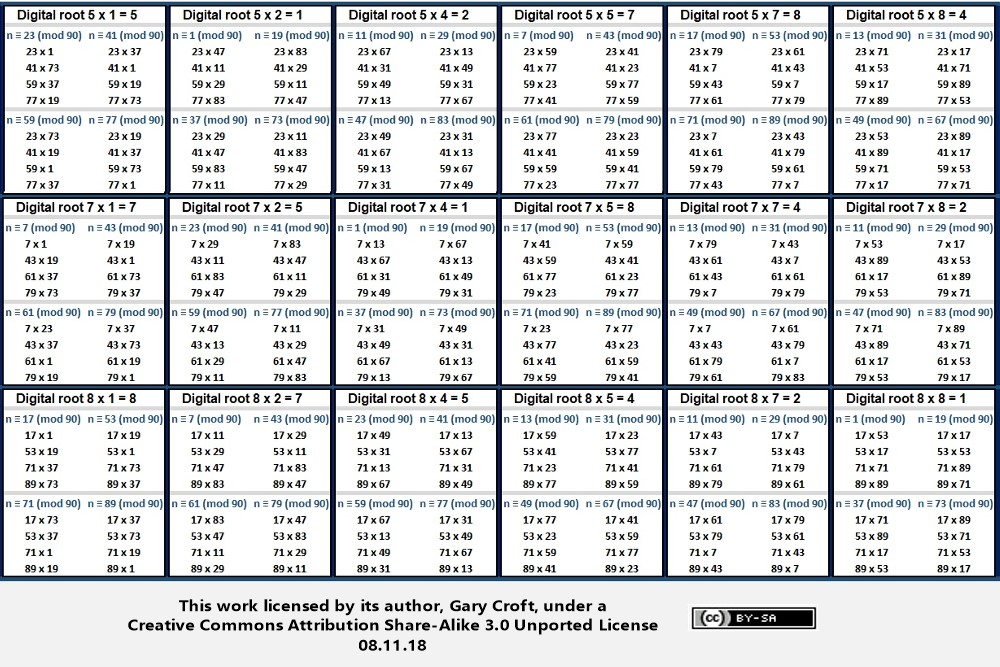### Modulo 90 Digital Root Multiplication/Factorization Matrix

Below is a modulo 90 multiplication matrix with the products converted to their digital roots which can be used in conjunction with the factorization matrices pictured above. The principal diagonal has been colored green to differentiate the squares:Studying the digital root of the squares highlighted in the matrix above we discover a period-24 palindrome,
{1, 4, 4, 7, 1, 1, 7, 4, 7, 1, 7, 4, 4, 7, 1, 7, 4, 7, 1, 1, 7, 4, 4, 1} {repeat ...},
and find the basis for a proof that the squares of all n ≡ {1, 7, 11, 13, 17, 19, 23, 29, 31, 37, 41, 43, 47, 49, 53, 59, 61, 67, 71, 73, 77, 79, 83, 89} modulo 90 (and therefore by definition all prime numbers > 5) distribute to digital roots 1, 4, or 7, categorized in three sets as follows:

n ≡ {1, 17, 19, 37, 53, 71, 73, 89}2 mod 90 = digital root 1
n ≡ {7, 11, 29, 43, 47, 61, 79, 83}2 mod 90 = digital root 4
n ≡ {13, 23, 31, 41, 49, 59, 67, 77}2 mod 90 = digital root 7

And note that all three sets have four bilateral 90-sums = 360. We've documented this sequence on the On-Line Encyclopedia of Integer Sequences; Ref: OEIS Sequence Number A240924.

Subsorting (sieving) the squares of twin prime candidates gives us:

n ≡ {1, 17, 19, 71, 73, 89}2 mod 90 = digital root 1
n ≡ {11, 29, 43, 47, 61, 79}2 mod 90 = digital root 4
n ≡ {13, 31, 41, 49, 59, 77}2 mod 90 = digital root 7

Each of the three sets in this sub-division has three bilateral 90-sums = 270.

And here we've color-coded the matrix to highlight digital root distribution symmetries:### Factorization Dyad Terminating Digit Patterns

The terminating digits of the factorization dyads we've been enumurating also have organizing principles that generate symmetry, as illustrated below (note the 16-digit palindrome):These symmetries can be traced to the period-24 terminating digit repetition cycle of our domain, each repetition possessing 12 bilateral-10 sums:

{1, 7, 1, 3, 7, 9, 3, 9, 1, 7, 1, 3, 7, 9, 3, 9, 1, 7, 1, 3, 7, 9, 3, 9} { repeat ... }

The simplest way to expose terminating digit symmetries is to matrix multiply them, as pictured below: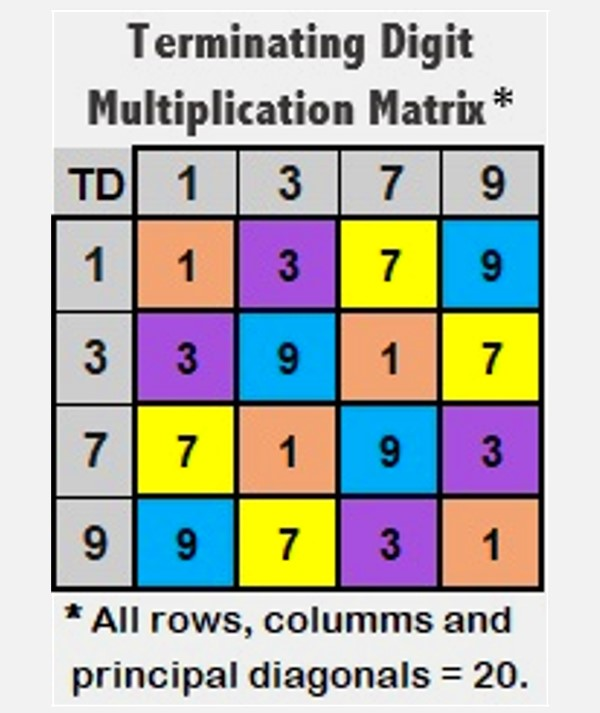### Modulo 90 Factorization: Congruence Dyad Matrix

A fascinating dimension of numbers ≡ {1, 7, 11, 13, 17, 19, 23, 29, 31, 37, 41, 43, 47, 49, 53, 59, 61, 67, 71, 73, 77, 79, 83, 89} modulo 90 is that modulo 90 congruence can be determined without division for any member of this sequence by matrixing a number's digital root with its terminating digit (see illustration, below). For example, take the number 389: Its digital root = 2 and its final digit = 9. Referencing the matrix below, we see that 389 is congruent to 29 mod 90.All Factor combinations for composite numbers within our modulo 90 defined domain can be rooted to one or more 'congruency dyads' drawn from the 24 candidates shown in the table above.

It can be easily proven that six of the digital root / terminating digit dyads in the matrix above classifying members of our domain cannot possibly be twin prime candidates for pairs > (5,7), given their closest proximity to other primes is ±4:

dr1...td7 (n ≡ {37} mod 90)
dr2...td3 (n ≡ {83} mod 90)
dr4...td7 (n ≡ {67} mod 90)
dr5...td3 (n ≡ {23} mod 90)
dr7...td7 (n ≡ {7} mod 90)
dr8...td3 (n ≡ {53} mod 90)

[Note: To quickly determine a number's modulo 90 congruence, reduce all digits preceding the number's terminating digit to their digital root. For example, the 1st three digits of 3797 reduce to digital root 1 leaving 17, and thus 2497 ≡ {17} mod 90. Remember that 9's are 'cast out' when calculating digital root.]

## The Prime Spiral Sieve as Star Birthing Chamber

Rotating Equilateral Triangles, Triangulation Mapping Matrices, Complex Polygons and the Digital Root of Modulo 90 Factorization and Twin Prime Candidate Sequencing in 24 Dimensions

"The search for symmetry, and the emotional pleasure we derive when we find it, must help us make sense of the world around us ... Symmetry is also economy. Symmetry is simplicity. Symmetry is elegance." From The Accidental Universe, by theoretical physicist Alan Lightman.

### Seeing Stars: Rotational Symmetry Groups, Modulo 90

When we stretch our modulo 30 ↔ 8-dimension horizon times 3, and look at the above factorization sequences from a modulo 90 ↔ 24-dimension digital root perspective, we make a profound discovery (illustrated in the graphics that follow): Straight out of group theory comes a triad of rotating equilateral triangles, ({1,4,7}, {2,5,8}, {3,6,9}), algorithmically permutated to produce 242 = 576 {9/3} star polygons per one complete cycle, which equates to 242 x 3 = 1728 rotating equilateral triangles possessing a total of 9 x 576 = 5184 vertices (and we note an amazing connection to 5184 in that there are exactly 1000 prime numbers between 1 and 892 and 82 * 92 = 5184, not to mention 82 * 9 = 576!). Each star's sequence of rotations is digitally rooted in a matrix acting as a correspondent between sets of geometric coordinates. These digital root sequences and their associated geometries are infinitely repeating (modulo 90), and the symmetries they create are simultaneously reflectional, rotational and translational.

We can say with certainty that the factorization algorithms for numbers not divisible by 2, 3, and 5 are complex polygon generators. Put more poetically perhaps, the Prime Spiral Sieve, when telescoped x 3 to modulo 90, is a star birthing chamber. These symmetry groups are digitally rooted in numbers ≡ to {1, 7, 11, 13, 17, 19, 23, 29, 31, 37, 41, 43, 47, 49, 53, 59, 61, 67, 71, 73, 77, 79, 83, 89} modulo 90, and form the basis of the 24 x 24 " Magic Mirror Matrix" (see also section below titled "Cirque de Primes Juxtaposed with the Magic Mirror Matrix").

Mathematicians often compare prime numbers to 'atoms' and composite numbers to 'molecules.' One could argue that it's consistent with this analogy to call digital root equilateral triangles {1,4,7} {2,5,8} the 'quark triads' of the number universe. In both cases we have a fundamental dyad of interacting triads. With regard to our modulo 90 triangulations, {1,4,7} and {2,5,8}, each has 3! = 6 possible rotational symmetry operations referenceable to the values of vertices 1 thru 9 of a {9/3} star polygon; thus we get: {1,4,7}, {1,7,4}, {4,1,7}, {7,1,4}, {4,7,1}, {7,4,1} vs. {2,5,8}, {2,8,5}, {5,2,8}, {8,2,5}, {5,8,2}, {8,5,2} (which you'll note we've ordered palindromically). These triangles interact combinatorially via digital root dyad sums to create {3,6,9}'s six possible rotational permutations {3,6,9}, {3,9,6}, {6,3,9}, {9,3,6}, {6,9,3} and {9,6,3}, illustrated below: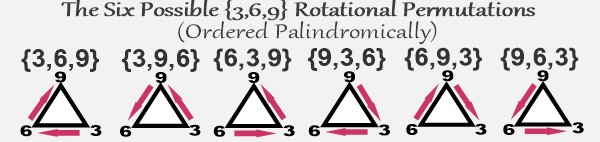As we've noted previously, the {3,6,9}'s triangular permutations provide fundamental structure to our domain in the form of repeating modulo 30, 60, 90 {3,6,9} cycles ... These progressions appear to be isomorphic to the geometric hierarchy of star polygons constructed from equilateral triangles such that (Schäfli notation in braces): {3/1}, or simply {3}, is to modulo 30 as {6/2} is to modulo 60 as {9/3} is to modulo 90; and this progression builds to infinity.

In much the same way that a Buddhist might say "It's the interaction between Yin and Yang that moves fate forward," we're arguing that it's the interaction between {1,4,7} and {2,5,8} that moves structure foward shaped by {3,6,9}'s six combinatorial symmetries. Writing about the importance of group theory developed by Évariste Galois, well known mathematician Marcus du Sautoy captures this idea in his book Symmetry: A Journey into the Patterns of Nature: "[Galois] discovered that it is the interactions between the symmetries in a group that encapsulate the essential qualities of the symmetry of an object."

[Note: Any argument for the significance of {3,6,9} must include genius inventor Nikola Tesla's famous quote: "If you only knew the magnificence of the 3, 6 and 9, then you would have the key to the universe."]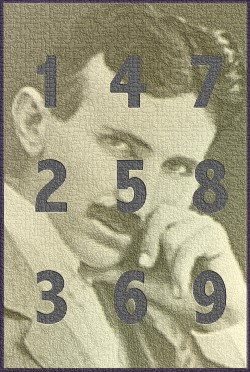Speaking of which, Tesla would have appreciated the patterns involving {3,6,9} structure illustrated in the table of sequences below, where we add the period-24 digital root of natural numbers not divisible by 2, 3 or 5 (oeis.org: A007775) to the period-32 palindromic digital root of Fibonacci numbers indexed to same (oeis.org: A227896).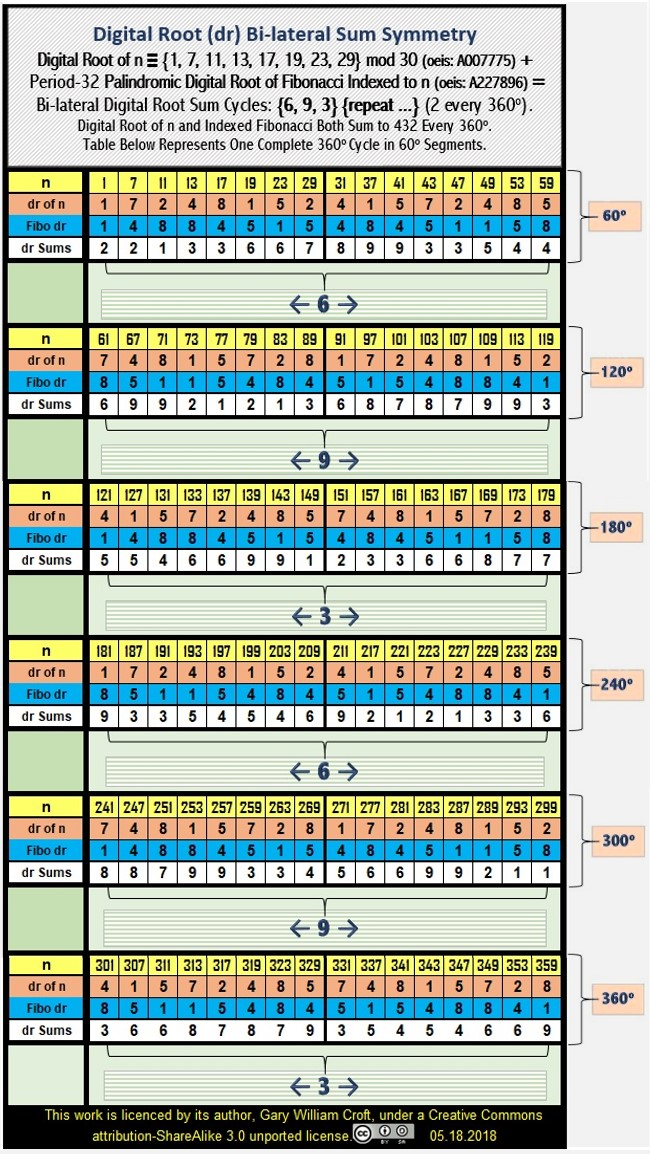Upon inspection, we find a repeating pattern of bilateral digital root sums that sequence in {6,9,3} cycles (2 every 360°). As we've noted in several places throughout this site, there are precisely 1000 prime numbers between 1 and 892. Interestingly, there are exactly 22 of these 360° cycles (equating to 44 of the {6,9,3} cycles illustrated above) between zero and 892, and the final term in the 22nd cycle is 7919, which happens to be the 1000th prime number. Also note that the digital root compiled for natural numbers congruent to {1, 7, 11, 13, 17, 19, 23, 29} mod 30 from 1 to 7919 = 9504 = 22 x 432 = 24 x 396 = 9 x 1056 (1056 being the number of pairs in our domain summing to 7920). The 1st and last terms in this 22 x 360° cycle block, 1 and 7919, sum to 7920 = 22 x 360. In addition (pun intended), 7919 + 7920 = 15840 = 44 x 360.

As we've noted elsewhere, the decimal expansion of 89's reciprocal can be derived additively from the Fibonacci sequence. Is it coincidental that the decimal expansion of 89's reciprocal is period-44, composed of 22 bi-lateral 9 sums = 198, and 7920/198 = 40, while 432 minus its reversal (234) = 198? And, amazingly, the first 89 digits of pi's decimal expansion sum to 432. (And dare we mention that 432 + its reversal = 666.)

And is it a coincidence that the index number of the 1000th prime number, as a natural number not divisible by 2, 3 or 5, is 2112? Not only is it palindromic, but it has the first 3 digits of the Fibonacci sequence overlapping in both positive and negative directions. Then consider that the 2112 elements in this range end-to-end possess perfect symmetry in the form of 1056 bilateral 7920 sums = 8363520 = 360 x 23232 ... 23232/2112 = 11, and 2112 has 11 at its center and its leading and final digits = 22. And, it's interesting to note, 8*3*6*3*5*2 = 4320 and 8363520/2112 = 3960 = 11*360, while 2*3*2*3*2 = 72, and the cumulative digital root from 1 to 72 = 360.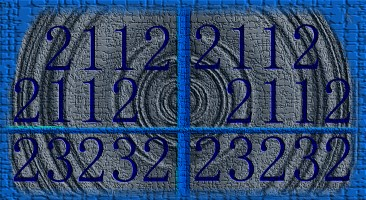And to cap it off, the three possible contiguous 2-digit numbers in 2112 (21, 12, 11) sum to 44. The mysterium tremendum of symmetries within symmetries.Oh, and by the way, the first 24 prime numbers, 2 + 3 + 5 + 7 + 11 + 13 + 17 + 19 + 23 + 29 + 31 + 37 + 41 + 43 + 47 + 53 + 59 + 61 + 67 + 71 + 73 + 79 + 83 + 89 = 963 = 3 * 321, while the average value of the 2112 elements in our domain from 1 to the 1000th prime number, 7919 = 3960 = 11 * 360. And 11 plus its digital root, 2, = 13. Not only are 11 and 13 the first twin prime pair in our domain but 11 + 13 = 24, the digital root periodicity of our domain, and 242 = 576, the number of modulo 90 factorization dyads and rotating {9/3} star polygons that determine the positioning of all composite numbers within our domain. Given the pervasiveness of palindromic prime 11 and its multiples in our schema, it would be fair to to call it the 'Fundamental Repunit.' It's the axe blow that splits a log perfectly down the middle with one fell swoop.

In toto, the numbers provide compelling evidence that the 1000th prime number is not randomly placed on the number line, but is in fact the perfectly positioned cornerstone of a rectangular shaped object with highly organized substructures dually enveloped by 892 and 7920. Study the relationships detailed below and you'll start to see a palindromic spine (viz., 11, 22, 33, 44, 55, 88, 121, 242, 343, 484, 2112, 23232), vertical and horizontal reflectional symmetry composed of prime numbers (especially, the 242 'Mirror Prime Pairs'), and other features that suggest this 'mathematical object' is worthy of further study. For example: The myriad ways that numbers 24 and its square, 576, play out dimensionally in this space are truly remarkable.

The diagram below captures the basic dimensions of this object, followed by a list of its many fascinating features. Then the matrix itself is presented, showing row and additive totals for primes (all primes hi-lited black). After that, you'll find a graphical "footprint" of the 484 prime numbers between 1 and 7920 (exactly 112 + 112 on each side!) that form 242 Mirror Prime Pairs.

[Note: There are several references below to 'cumulative digital root.' An explanation of this term along with formulae and examples can be found at the end of this section. Also, be warned that you'll find a certain amount of annoying redundancy in the list below. This was done deliberately to expose particularly interesting juxtapositions and associations. Also note that 576 has been 'boldened' to emphasize its importance. And one can't help but wonder if there's a connection between the 88-unit dimension of this object and the 88 pitches of a modern piano(?).]

Here's a quote to keep in mind while scanning these data:

"Mathematicians have long wondered at the haphazard way in which the primes are sprinkled along the number line from one to infinity, and universality offers a clue. Some think there may be a matrix underlying the Riemann zeta function that is complex and correlated enough to exhibit universality. Discovering such a matrix would have 'big implications' for finally understanding the distribution of the primes ..." Natalie Wolchover, "In Mysterious Pattern, Math and Nature Converge," Quanta Magazine, 02.05.2013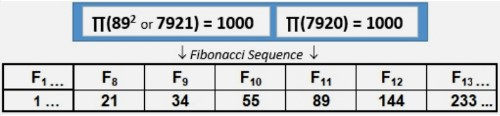1. F10 (55) x F12 (144) = 7920.
2. F10 (55) x F12 (144) - F1 (1) = 7919 (the 1000th prime number).
3. F10 (55) x F12 (144) + F1 (1) = 7921 = 892 (and every 11th Fibonacci number, starting with F11, has 89 as one of its divisors).
4. The additive sums of Fibonacci numbers sequentially divided by 10 raised to the power of their sequence numbers equate to the reciprocal of 89. Similarly, the additive sums of Fibonacci numbers divided by 10 raised to the power of 109 minus their index numbers produce the reciprocal of 109.
5. 2 x 32 x 109 - 2 x 32 x 89 = 360.
6. F11 (89). The decimal expansion of 89's reciprocal (1/89) is period-44, composed of 22 bi-lateral 9 sums = 198, and 7920/198 = 40.
7. Total value of the 24 * 88 = 2112 cell matrix is 8363520, and average value is 3960, while 3960/360 = 11..
8. 8 + 3 + 6 + 3 + 5 + 2 + 0 = 24.
9. 89's index # as a member of this domain = 24, while 89's square has index #2113 (a twin prime, with 2111).
10. 89/92 = 1.0987654321.
11. The first 89 digits to the right of the decimal point in pi sum to 432.
12. (89 * 91) - (89 + 91) = 7919.
13. 902 - 892 = 179 (prime); 902 - 7919 = 181 (prime); twin primes 179 + 181 = 360.
14. 89 and its reversal require the most reversals and additions to become palindromic (steps required = 24).
15. 9+8+7+6+5+4+3+2+1+2+3+4+5+6+7+8+9 = 89.
16. 89 is the 24th prime and 24th n not divisible by 2, 3, or 5.
17. F4 (3) + F8 (21) = 24.
18. The prime numbers between 1 and 24 (2, 3, 5, 7, 11, 13, 17, 19, 23) sum to 100, while the first 24 integers sum to 300.
19. 431, 433 are twin primes.
20. 7919 ≡ 431 (mod 576), while 7921 (or 892) ≡ 433 (mod 576), and, of course, 7920 ≡ 432 (mod 576).
21. F10 (55) + F14 (377) = 432.
22. 242 = 576.
23. F8 (21) + F9 (34) + F10 (55) + F11 (89) + F12 (144) + F13 (233) = 576.
24. 576 = number of modulo 90 factorization dyads and associated {9/3} star polygons that algorithmically account for all composite numbers > 5 not divisible by 2, 3, or 5. (Scroll up this page for a detailed account of these.)
25. 5 x 7 x 6 = 210 = 2 x 3 x 5 x 7 (the 4th primorial).
26. 576 + its cumulative digital root (2880) = 3456.
27. 3 x 4 x 5 x 6 = 360.
28. F24 = 46368.
29. 4 x 6 x 3 x 6 x 8 = 3456.
30. The cumulative sum of natural numbers {1, 2, 3, ..} from 1 to 2112 (Index # of the 1000th prime) = 2231328. (Here are the equations for the cumulative sums (k = 1,2,3...n) of natural numbers, bifurcated by odd vs. even:
For odd n: k = (n +1)/2 * n. For even n: k = n/2 * (n +1).)
31. 2 x 2 x 3 x 1 x 3 x 2 x 8 = 576.
32. 576 = number of 4 x 4 Latin Squares.
33. 2112/(4 x 4) = 132; 3 x 132 = 396; 8363520/3960 = 2112; 8363520/132/30 = 2112; 8363520/(2112 x 360) = 11.
34. In 2016, Ukrainian mathematician Maryna Viazovska published two papers (the 2nd in collaboration with associates) proving that the densest packing of congruent spheres in Euclidean space is in dimensions 8 and 24; here's the theorem statement for the latter: "Theorem 1.1. The Leech lattice achieves the optimal sphere packing density in R24, and it is the only periodic packing in R24 with that density, up to scaling and isometries." Heres a link to the 2nd paper: "The sphere packing problem in dimension 24".
35. The 576th prime member of this domain, 4211, has index # 1123 (1st 4 Fibonacci numbers).
36. Cumulative digital root sequence (1 - 7920) contains 345 prime numbers.
37. 345's divisors (1, 3, 5, 15, 23, 69, 115, 345) = 576.
38. 57610 = 48411.
39. 484 = number of primes in range (1 - 7920) that form 242 'Mirror Prime Pairs.'*
* prime pairs in each period-24 segment of our domain with summands that spatially 'mirror' opposite the center-line of a 24-wide matrix. All such pairs are evenly divisible by 90. For example, the first row contains 9 such pairs (working from the center outward):

(43 + 47); (37 + 53); (31 + 59); (29 + 61); (23 + 67); (19 + 71); (17 + 73); (11 + 79); and (7 + 83)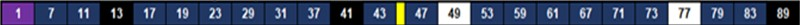(Scroll down to find a "footprint" of all 242 of these pairs.)

40. 8363520/242 = 34560 = 96 x 360 = 80 x 432 = 60 x 576.
41. 8363520/96 = 242 x 360. (96 is the 360° periodicity of terms for n not divisible by 2, 3, or 5.)
42. 8363520/360 = 23232
43. 23232/360 x 30/8 = 242. (30/8 is ratio of natural numbers 1, 2, 3, ... to n not divisible by 2, 3, or 5).
44. A day on Venus is equivalent to 242 Earth days. 365 - 242 = 123.
45. Entire matrix consists of 1056 dyads summing to 7920. Of these, 243 form prime pairs. 243 = 92 + 92 + 92 = 35.
46. Cumulative natural number sum (1 - 7920) = 31367160 = 11 x 892 x 360 = 22 x 5 x 892 x 198.
47. Cumulative digital root of 7920 = 39600, while cumulative sum n {1, 2, 3, ...} (1 - 7919) = 31359240 = 7919 * 3960.
48. 39600/360 = 110.
49. Our mathematical object, a matrix, is 24 x 88 = 2112 cells.
50. 2112/(8/30) = 7920.
51. 2112/(88/24) = 576.
52. 2112/82 = 33.
53. (2112/82) - (576/82) = 24.
54. 2112 = 26 x 3 x 11.
55. 2112/360 x 30/8 = 22 ... 2112/90 x 30/8 = 88.
56. 2112 is the sum of 2 distinct powers of 2, i.e., 26 + 211 = 2112 (ref. oeis.org: A018900).
57. 2112 is a 4 times triangular number, defined as a(n) = 2 * n * n * (n+1),
or 2 * 2112 = 4224; 4224 * 2112 = 8921088 = 576 * 27 * 112; 8921088 * (2112 + 1) = 18850258944 = 576 * 27 * 112 * 2113 (ref. oeis.org: A046092).
58. 2111, 2113 are twin primes
59. 2112's aliquot sum (the sum of its divisors) = 3984.
60. 3 x 9 x 8 x 4 = 432 x 2.
61. 2112/96 = 242 (242 = number of Mirror Prime Pairs and 96 is the 360 degree digital root Fibonacci periodicity when indexed to n not divisible by 2, 3, or 5, i.e., period 32 every 120 degrees).
62. 8363520/576 = 14520.
63. 14520/60 = 242
64. 8363520/242 = 34560.
65. 3 * 4 * 5 * 6 = 360.
66. 34560 is the 6th "superfactorial," i.e., the product of first 6 factorials, viz. 1! * 2! * 3! * 4! * 5! * 6! = 34560 (ref: oeis.org: A000178). Curiously, the 7th superfactorial (24883200) = 576 * 432 * 100.
67. 34560/360 = 96.
68. 34560/432 = 80.
69. 34560/576 = 60.
70. 8363520 = 22 * 3 * 5 * 242 * 576.
71. 8 * 3 * 6 * 3 * 5 * 2 = 4320 = 10 * 432 = 12 * 360.
72. 8363520/360 = 23232
73. 8363520 = 112 * 192 * 360.
74. 23232/2112 = 11.
75. 23232/(8/30) = 242 * 360.
76. 2112/192 = 11.
77. 2112 ≡ 192 * 2 (mod 576).
78. 2112/192 = 11.
79. (191,193) are twin primes; 191 + 192 + 193 = 576.
80. 8363520/192 = 43560 = 112 * 360.
81. 192 = total number of partitions of 10.
82. 192 = 5 + 7 + 11 + 13 + 17 + 19 +23 + 29 + 31 + 37 (10 consecutive primes).
83. 8363520 = 96 x 242 x 360.
84. 8363520 = 80 x 242 x 432.
85. 8363520 = 27 x 33 x 5 x 484.
86. 8363520 = 60 x 242 x 576.
87. 8353520 x 30/8 = 31363200 = 242 x 3602.
88. 8363520 x 30/8 + 3960 = cumulative sum (1 - 7920) = 31367160; 3 x 1 x 3 x 6 x 7 x 1 x 6 = 567 x 4.
89. 31367160 = 360 x 11 x 892.
90. Average value of the 2112 elements in our domain from 1 to the 1000th prime number, 7919 = 3960 and 3960/360 = 11.
91. 1056 (number of dyads summing to 7920) x 8/30 (ratio of numbers not divisible by 2, 3, or 5 to natural numbers, 1, 2, 3, ...) = 3960 (the average value of matrix).
92. Cumulative digital root compiled for natural numbers congruent to {1, 7, 11, 13, 17, 19, 23, 29} mod 30 from 1 to the 1000th prime, 7919 = 9504.
93. 9504 = 22 x 432 = 24 x 396 = 88 x 108 = 25 x 33 x 11.
94. 9504 = 66 x 144, while 6 x 6 x 1 x 4 x 4 = 576.
95. 9504/1056 (number of pairs in our domain summing to 7920) = 9.
96. 8363520/9504 = 880.
97.98. 7 x 9 x 1 x 9 = 567.
99. Of the 1000 primes between 1 and 7920, 997 are n not divisible by 2, 3, or 5 ... 9 x 9 x 7 = 567.
100. In physics, 11 is the number of dimensions in M-theory "that unifies all consistent versions of superstring theory."
101. 11 is not only the Fundamental Repunit. It's also the Fundamental Dyad (1 + 1 = 2) and the Fundamental Palindrome! And, of course, it's also the first palindromic prime and 1+1=2 ... initiates the Fibonacci sequence. And should we be surprised that the 'Magic Angle' for superconductivity (a precipitous drop in electrical resistance) when two sheets of graphene are stacked and twisted at a relative angle, is 1.1°! Lastly, when we add 11 + 89, we score 100!

Here is the matrix under discussion: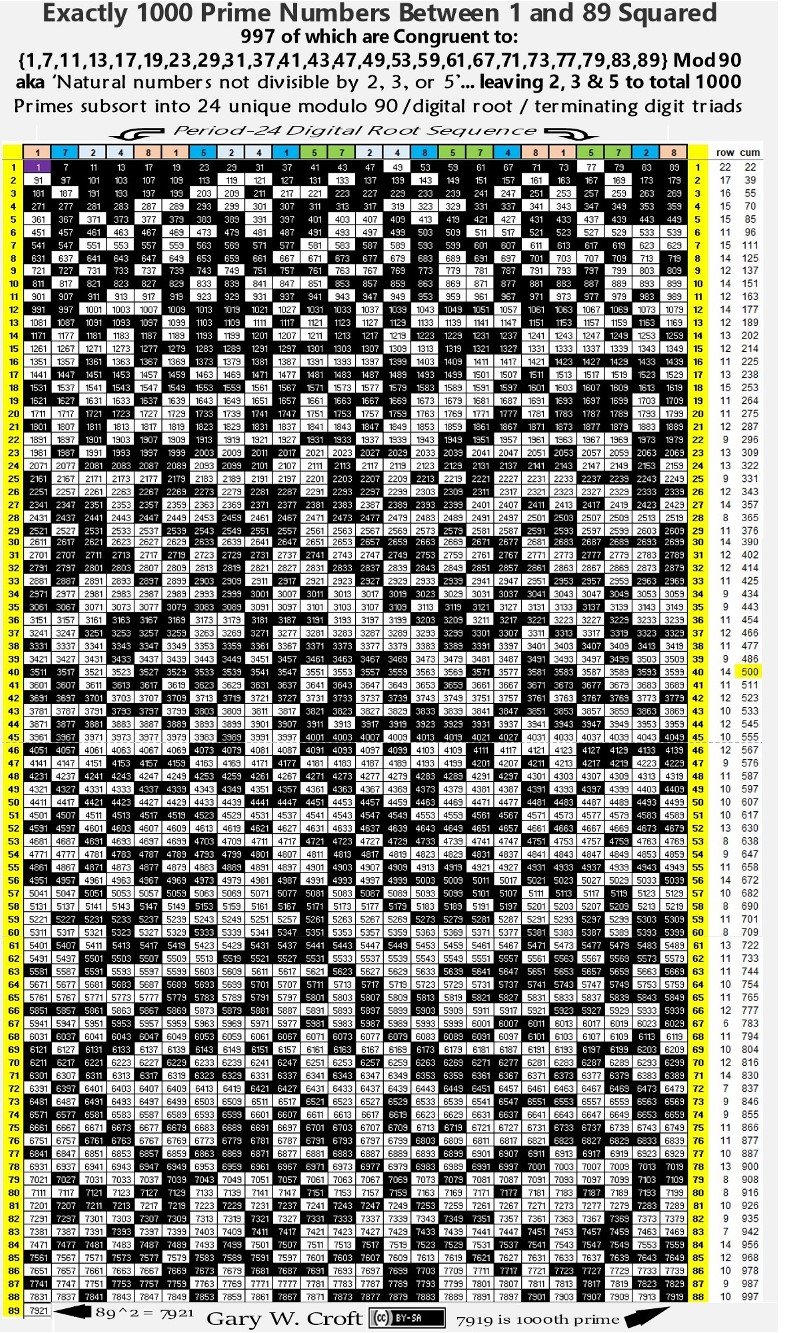Below is an image reflecting the "Mirror Prime Pairs" referenced above. Astounding that each side of the matrix has 112 + 112 = 242 of these primes: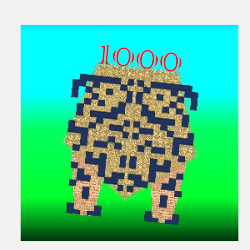The following formulae calculate cumulative digital root, 1, 2, 3 ... n. They are effectively 'if-then' statements: If digital root n, then apply appropriate equation (and note that the string of 'corrections' forms a palindrome).

Cumulative Digital Root (cumdr = k) for natural numbers (n) 1, 2, 3, ...

For dr n = 1: k = 5n - 4; 〈e.g., n = 37: k = 5 * 37 - 4 = 181〉.

For dr n = 2: k = 5n - 7; 〈e.g., n = 29: k = 5 * 29 - 7 = 138〉.

For dr n = 3: k = 5n - 9; 〈e.g., n = 30: k = 5 * 30 - 9 = 141〉.

For dr n = 4: k = 5n - 10; 〈e.g., n = 67: k = 5 * 67 - 10 = 325〉.

For dr n = 5: k = 5n - 10; 〈e.g., n = 23: k = 5 * 23 - 10 = 105〉.

For dr n = 6: k = 5n - 9; 〈e.g., n = 24: k = 5 * 24 - 9 = 111〉.

For dr n = 7: k = 5n - 7; 〈e.g., n = 16: k = 5 * 16 - 7 = 73〉.

For dr n = 8: k = 5n - 4; 〈e.g., n = 17: k = 5 * 17 - 4 = 81〉.

For dr n = 9: k = 5n - 0; 〈e.g., n = 72: k = 5 * 72 - 0 = 360〉.

The examples above were taken from the section of spreadsheet cut-and-pasted below covering the range of natural numbers 1 thru 72 (and associated cumulative digital root 1 thru 360). Prime numbers are hi-lited in yellow.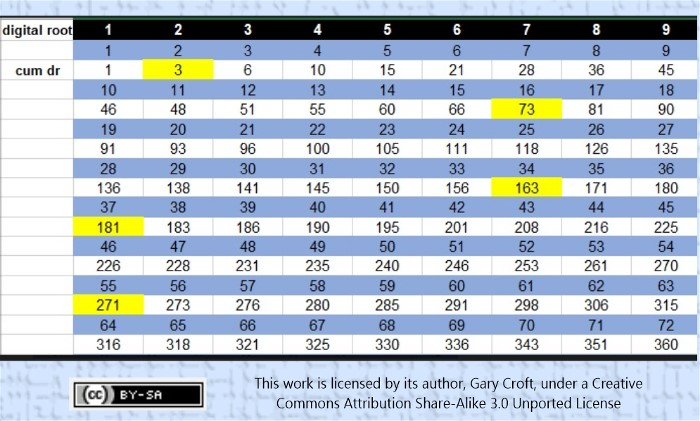Based on this analysis, it can easily be proven that, with the exception of the first prime (1+2=3) all primes in this sequence are congruent to {1 or 73} modulo 90, and therefore all, with the exception of number 3, have digital root 1. And, as we stated earlier, numbers 1 thru 7920 generate 345 such 'cumulative digital root' primes (and of these, 171 are n ≡ {1} mod 90 and 173 are n ≡ {73} mod 90. Number 3 is the only outlier).

More convoluted, is the manifold formula for the cumulative digital root of n ≡ {1,7,11,13,17,19,23,29} mod 30 (aka, natural numbers not divisible by 2, 3, or 5). The cumulative sums of the first 24 digital roots establish 'starting block' values, employed as 'additives' in the cascading formulations that follow. Because the modulus is synchronized with its period-24/modulo90 digital root, with the 1st row (and every succeeding row) having an additive sum of 108, every column also increments in +108 intervals.

For example, to calculate the cumulative digital root from 1, ... 7919 (the 1000th prime): Given 7919 ≡ {89} mod 90, we calculate as follows: 7919/90 =  [whole # part taken]; 87 * 108 + 108 = 9504. The [n/90] [whole number part taken] calculations give column interval multipliers. Note that the whole number parts taken for the first 24 terms = zero, leaving only the baseline 'additives.'### Period 24 In All Directions

We stated earlier that this is a 24-dimension model. After putting in the proverbial 10,000 hours studying '24-beat' patternization, we've come to the conclusion that period-24 is the key to the "Theory of Everything" and that a hypothetical E24 Petrie Projection will one day loom large as E8 is understood to be the leg of a triad, with E16, leading to E24; the three being analogous to:

Mod 30 → E8 → {3} star polygon ...

Mod 60 → E16 → {6/2} star polygon ...

Mod 90 → E24 → {9/3} star polygon ...

... building geometrically to infinity ...

We've dubbed this 'The Theory of Everything ... but the Kitchen Sink,' illustrated below: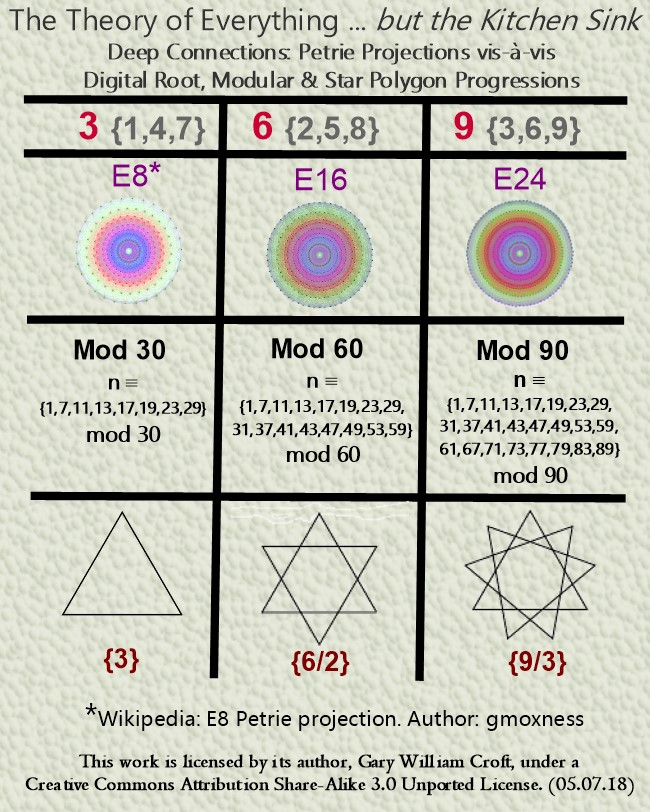Explore the incredible symmetries that come into focus when the lense aperature, so to speak, of the Prime Spiral Sieve is tripled to modulo 90, synchronizing its modulus with its period-24 digital root, and perhaps you'll see why we make this bold assertion. The mathematical balancing and resolution of this domain, which correlates with a hypothetical E24, including structures that determine the distribution of prime numbers, are fundamentally period-24.

Aside from each of the 24 deterministic modulo 90 factorization progressions having a repetition cycle of 24, there is a hidden dimension generated by successive digital root dyads for each factorization sequence that is also period-24 given that (and there's a proof for this): Any initial dyad of non-zero single-digit numbers enters a periodic cycle of length-24, except for eight pairs that enter cycles of length-8, {(3,3), (3,6), (3,9), (6,3), (6,6), (6,9), (9,3), (9,6)}, and one dyad, (9,9), which is periodic of length-1. (Source: Adapted from a comment posted on the On-Line Encyclopedia of Integer Sequences: OEIS #A030132: Digital Root of Fibonacci(n)). For example, the first two terms of the Fibonacci digital root sequence just referenced, (1,1), enter a periodic cycle of length-24, as follows:

{1, 1, 2, 3, 5, 8, 4, 3, 7, 1, 8, 9, 8, 8, 7, 6, 4, 1, 5, 6, 2, 8, 1, 9} {repeat ... }.

Our modulo 90 framed domain, n ≡ to {1, 7, 11, 13, 17, 19, 23, 29, 31, 37, 41, 43, 47, 49, 53, 59, 61, 67, 71, 73, 77, 79, 83, 89} mod 90, also has a period-24 digital root:

{1, 7, 2, 4, 8, 1, 5, 2, 4, 1, 5, 7, 2, 4, 8, 5, 7, 4, 8, 1, 5, 7, 2, 8} {repeat ...}.

The twenty-four period-24 digital root factorization sequences derived from our domain can be parsed into six (6) digital root sub-sets, each consisting of 4 elements, that roll up under digital roots 1, 2, 4, 5, 7, 8, as follows (noting that all sequences for the first complete factorization round initialize with n x 7; the 1st and 2nd rounds are demarcated by 912 and the 2nd round initializes with 91 x 97. Further down this page you'll find a link to a spreadsheet with tables that make these sequences and their transitions clear.):

Numbers ≡ {1, 19, 37, 73} mod 90 (i.e., digital root = 1) sequence as:
{7, 2, 4, 8, 1, 5, 2, 4, 1, 5, 7, 2, 4, 8, 5, 7, 4, 8, 1, 5, 7, 2, 8, 1} {repeat ... }
Numbers ≡ {11, 29, 47, 83} mod 90 (i.e., digital root = 2) sequence as:
{5, 4, 8, 7, 2, 1, 4, 8, 2, 1, 5, 4, 8, 7, 1, 5, 8, 7, 2, 1, 5, 4, 7, 2} {repeat … }
Numbers ≡ {13, 31, 49, 67} mod 90 (i.e., digital root = 4) sequence as:
{1, 8, 7, 5, 4, 2, 8, 7, 4, 2, 1, 8, 7, 5, 2, 1, 7, 5, 4, 2, 1, 8, 5, 4} {repeat … }
Numbers ≡ {23, 41, 59, 77} mod 90 (i.e., digital root = 5) sequence as:
{8, 1, 2, 4, 5, 7, 1, 2, 5, 7, 8, 1, 2, 4, 7, 8, 2, 4, 5, 7, 8, 1, 4, 5} {repeat … }
Numbers ≡ {7, 43, 61, 79} mod 90 (i.e., digital root = 7) sequence as:
{4, 5, 1, 2, 7, 8, 5, 1, 7, 8, 4, 5, 1, 2, 8, 4, 1, 2, 7, 8, 4, 5, 2, 7} {repeat … }
Numbers ≡ {17, 53, 71, 89} mod 90 (i.e., digital root = 8) sequence as:
{2, 7, 5, 1, 8, 4, 7, 5, 8, 4, 2, 7, 5, 1, 4, 2, 5, 1, 8, 4, 2, 7, 1, 8} {repeat … }

Take a closer look at the above sequences, and you'll detect the perfect bilateral 9-sum and diagonal 90-sum symmetry hi-lited below: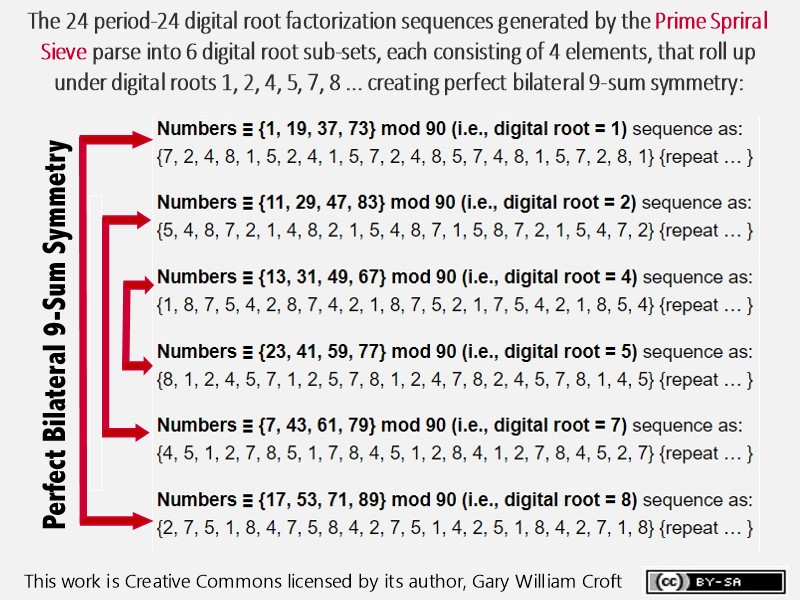The color-coded matrices pictured below illustrate that, when you sequentially align the twenty-four period-24 digital root sequences, perfect symmetry is revealed, in that every row has 12 bilateral 9 sums working from the outside in (or inside-out), and the additive sum of every row and column is 108. The principal diagonals also have 12 bilateral 9 sums, albeit in a cross-diagonal manner, summing to 75 + 141 = 216 = 108 x 2.Below are shown two of the 24 sets of period-24 digital root factorization sequences listed above i.e., the sequences of numbers congruent to {7} and {11} modulo 90 sequentially multiplied times numbers congruent to {1, 7, 11, 13, 17, 19, 23, 29, 31, 37, 41, 43, 47, 49, 53, 59, 61, 67, 71, 73, 77, 79, 83, 89} modulo 90, starting with 7. In addition to modulo 90, we display modulo 30 patterns and the interesting way they parse with repeating bilateral-30 sum symmetries. To the right of each digital root sequence we demonstrate how they generate rotating triangulations ({1,4,7}, {2,5,8}, {3,6,9}) in the form of 3 x 3 matrices which in turn can be extrapolated into {9/3} star polygons: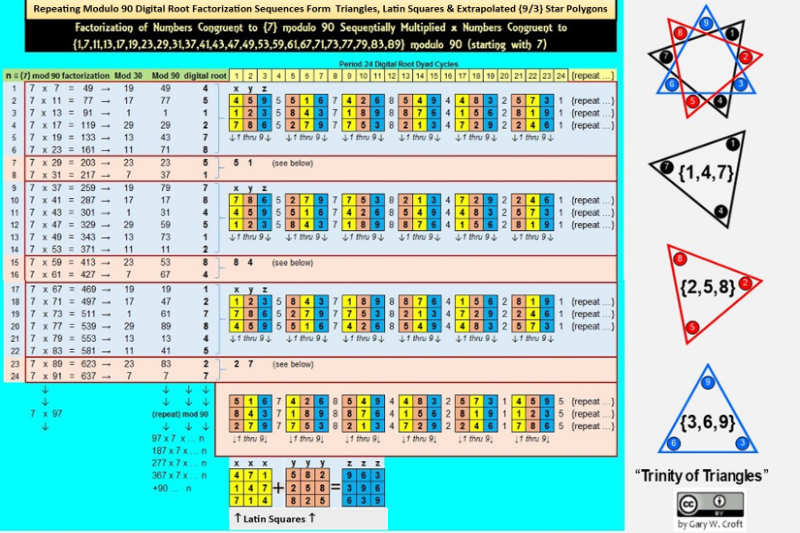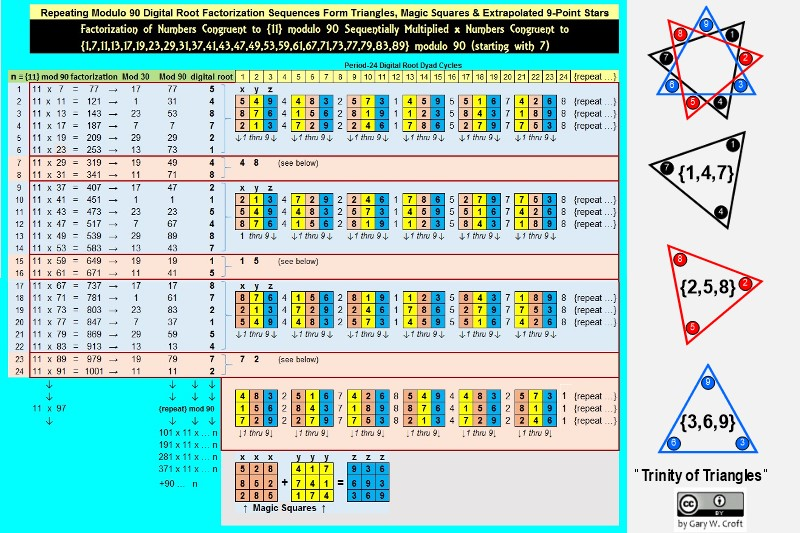Scanning the factorization products in the two examples above, we can quickly determine which of them impact twin prime candidates, given all twin primes greater than (5,7) are congruent to either {11,13}, {17,19} or {29, 1} modulo 30. For example, for n congruent to {7} modulo 90 factorization configurations pictured above, we see that exactly 18 of the 24 products (or 75%), index (identify) composite twin prime candidates (shown in brackets): 47 , , 79, 89 ,  121, 131 ,  163, 257 ,  289, 299 ,  331, 341 ,  373, 467 ,  499, 509 ,  541, 551 , and  583. Note how the lower vs. higher valued products alternate with each pair of twin prime candidates.

The remaining 6 products, of course, identify "isolated" prime candidates that are composite. We go into greater depth regarding digital root dyad cycles specifically related to twin primes under the headings "Digital Root Factorization Dyads of the Twin Prime Distribution Channels" and "Twin Prime Magic Squares," toward the bottom of this page.

To access an Excel spreadsheet showing the derivation of all 24 digital root factorization sequences and their corresponding period-24 matrix cycles, click here: Digital_Factorization_Roots_24x24_Matrix.xlsx.

Tables within the afore-linked spreadsheet reveal how the 24 infinitely repeating period-24 sequences each generates 24 matrices that extrapolate to {9/3} star polygons for a total of 24 x 24 = 576 = 9 x 82 9-pointed star polygons per complete factorization cycle. [Note: This count excludes the 'Fibo Bounds' described in the Note following this paragraph.] Also revealed are beautiful mod 30 vs. mod 90 symmetries. For example, comparing the digital root sequences for numbers congruent to {1} vs. {29} mod 30 and/or {1} vs. {89} mod 90 exposes beautiful counter-balancing symmetries.

[Note: Numbers ≡ {1} modulo 90 squared are segregated from the sets of 24x24 factorization sequences. Namely, 1x1, 91x91, 181x181, ... create a 'supra-sequence' of demarcating squares directly before and after each of the complete factorization sets of 24. For example, 1x1 and 91x91 act as bookends before 1x7 and after 89x91 (which in turn draws a line before 91x97). Poetically–if not profoundly–numbers ≡ {1} modulo 90 squared have other dimensions of 'oneness' when you consider that their digital roots and terminating digits also equal One.]

At the heart of 24-beat periodicity is the fundamental structure pictured below, showing the relationship between 24 and 108, the latter a quartile leg of 432 (another number of great import, as we discuss elsewhere on this site). Note the 'star' symmetry of the 12 lateral 9 sums.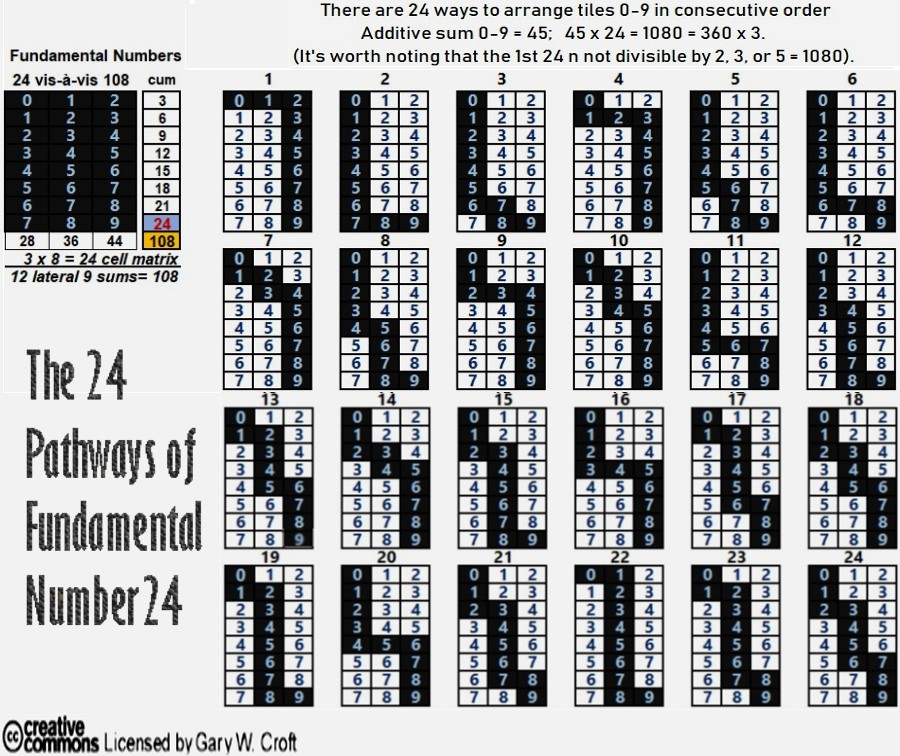### Beautiful 'Palindromagon' Constructed from Twin Prime Digital Root Dyad Cycles

And now to pull the proverbial rabbit out of the hat. Above we demonstrated that when sequenced vertically and grouped in sets of three pairs, the period-24 digital root dyad cycles generated by factorization algorithms produce permutating equilateral triangles in the form of 3 x 3 matrices from which can be extrapolated {9/3} star polygons.

An obvious question: What happens when the generator cycles are sequenced horizontally? The answer, it turns out, is pleasing to both mind and eye.

For discussion sake, we'll focus on twin primes. They, like the factorization algorithms discussed above, are permutated via 3 x 3 matrices populated by numbers 1 thru 9 and initiated (generated) by a trio of dyads involving digital roots 1, 2, 4, 5, 7, 8, which–when sequenced algorithmically–combinatorially form elegant 'geo-arithmetric' symmetry groups.

Elsewhere on this site we published a "Proof by Construction for the Digital Root Sequencing of Twin Primes" demonstrating that all twin prime pairs greater than (5,7), i.e., starting with (11,13) must have digital root sequencing of either {2,4}, {5,7} or {8,1}. A perfect example of such cascading dyads would be the first three prime twinnings of numbers congruent to {11,13} modulo 30: {11,13 = digital root 2 + 4 = 6} + 30 {41,43 = digital root 5 + 7 = 3} + 30 {71,73 = digital root 8 + 1 = 9} ...

When we additively sum the three period-24 digital root cycles these dyads produce, then tier them, we create six 3 x 3 matrices (each containing values 1 thru 9) separated by repetitive number tiers in the following order: {1,1,1} {5,5,5} {7,7,7} {8,8,8} {4,4,4} {2,2,2}. The six matrices these tiers demarcate are the source of triangular coordinates when translated into vertices of a modulo 9 circle (which by definition has 9 equidistant points around its circumference, each separated by 40°).

The series of diagrams below show the six geometric stages culminating in a complex polygon of extraordinary beauty. We've dubbed this object a 'palindromagon' given that the coordinates of the 18 triangulations produced by the digital root dyadic cycles in the order sequenced sum to a palindrome: 639 693 963 369 396 936. Remarkably, this periodic palindrome, with additive sum of 108, sequences the 6 possible permutations of values {3,6,9}. Interesting to consider a geometric object with a hidden palindromic dimension. But that's not all: When the six triadic permutations forming the palindrome are labeled A, B, C, D, E, F in the order generated, ACE and BDF form 3 x 3 Latin squares. In both cases all rows, columns and principal diagonals sum to 18:

ACE ... BDF
693 ... 639
369 ... 963
936 ... 396

The output of these algorithmically sequenced triangulations is fundamentally a geometric representation of the twin prime distribution channels (and, as we noted above, the same geometry is expressed in factorization sequencing, albeit the vertices may be ordered differently. This is because each set of three generator dyads roots to the same six elements: 1, 2, 4, 5, 7, 8. Thus, for example, dyad sets ({1,2} {4,5} {7,8}) and ({2,4} {5,7} {8,1}) will generate identical complex polygons, despite their vertices being sequenced in different orders.).

It's remarkable that objects consisting of star polygons, spiraling irregular pentagons, and possessing nonagon perimeters and centers, can be constructed from only 27 coordinates pointing to 9 triangles in 3 variations. Each period-24 cycle produces two 'palindromagons,' as illustrated below: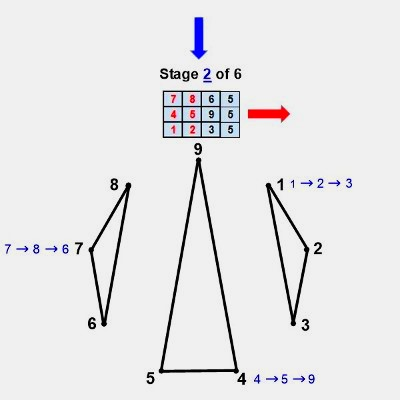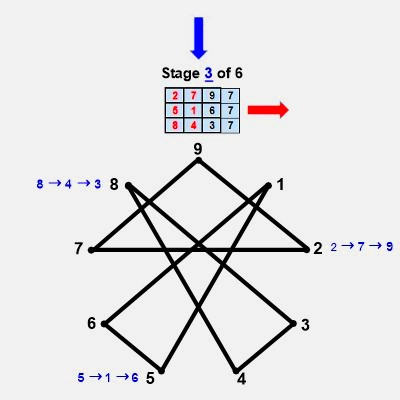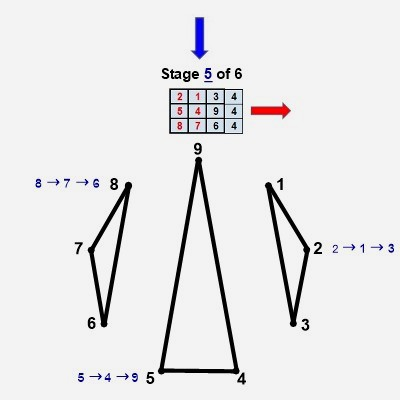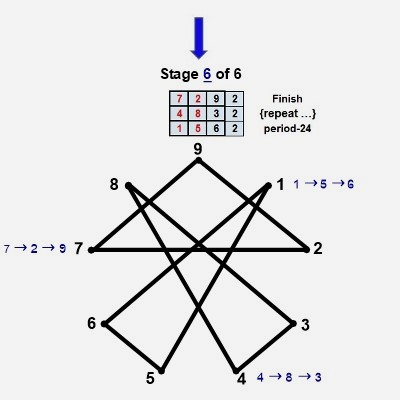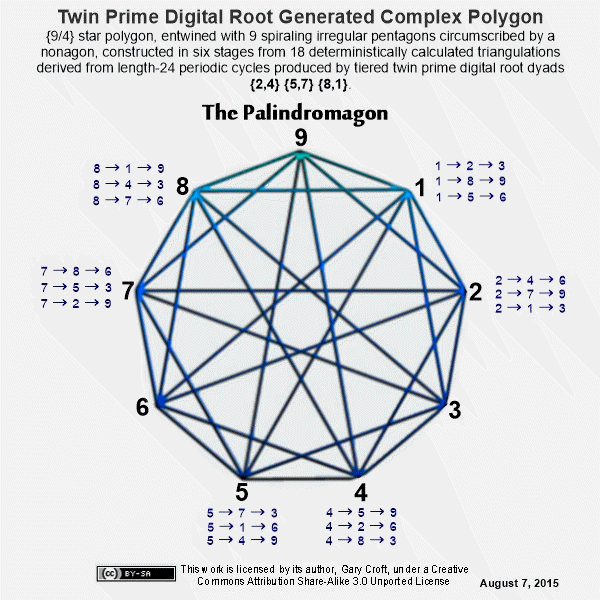Next we combine the vertical triangulations from our digital root cycles forming {9/3} star polygons with the horizontally generated Palindromagon, and discover that the consolidated object is one well known to geometers: It's a Regular enneazetton (8-simplex) (or a 9-faceted polytope in 8 dimensions), which, according to Wikipedia, contains: 9 vertices, 36 edges, 84 triangle faces, 126 tetrahedron cells, 126 5-cell 4-faces, 84 5-simplex 5-faces, 36 6-simplex 6-faces, and 9 7-simplex 7-faces.Lastly, let's compare the above-pictured 'enneazetton' to an 18-gon 9-point star generated by the first three primes; 2, 3 and 5 (pictured below), and we see that they are identical, save for the number of sides (9 vs. 18). They are essentially convex and concave versions of each other. This is geometric confirmation of the deep if not profound connection between the three twin prime distribution channels (which remember have 2, 3, and 5 encoded in their Prime Spiral Sieve angles) and the first three primes, 2, 3, and 5:### Additive Compilation of the Twin Prime Candidates

From what we learned above about segregating twin prime candidates, we can demonstrate that they compile additively in perfect progression, completing an infinite sequence of circles (multiples of 30 and 360):We've multiplied the twin prime candidate pair sums by 15° to show the equivalent arc of rotation of each pair in a modulo 90/24-element per rotation spiral, where 1 = 15° and 24 x 15 = 360°. Thus, when you divide the products by 360°, you get circular equivalents, e.g., 11+13=24; 24x15=360; 360/360=1 (thus, the 1st pair in the first thirt is equivalent to one 360° rotation).

### Cirque de Primes

A thirt, in case you're wondering, is a useful unit of measure when discussing intervals in natural numbers not divisible by 2, 3 or 5. A thirt, equivalent to one rotation around the Prime Spiral Sieve is like a mile marker on the prime number highway. If we take the Modulo 30 Prime Spiral Sieve and expand it to Modulo 360, we see that there are 12 thirts in one complete circle, or 'cirque' as we've dubbed it. Each thirt consists of 8 elements. The first two thirts are {1,7,11,13,17,19,23,29} and {31,37,41,43,47,49,53,59}. The last element in thirt1, 29, and the 1st element in thirt2, 31, are rooted in -1 and 1, respectively: where -1+30=29 and 1+30=31. These are twin primes, followed by an infinite sequence in intervals of 30 of twin prime candidates, that serve as the center stripes of the prime number highway, and thus: {-1,+1} +30 {29,31} +30 {59,61} +30 {89,91} +30 {119,121} +30 {149,151} +30 {179,181} +30 {209,211} +30 {239,241} +30 {269,271} +30 {299,301} +30 {329,331} +30 {359,361} completes cirque1, albeit, paradoxically, 361 is the start of cirque2. You can say that {359,361} straddle the 1st and 2nd cirques exactly at 360°, just as {719,721} straddle cirque2 and cirque3 at 720 (or 360x2) ... This is congruent with the fact that all repeating patterns at the digital root level, including the digital roots of Fibonnaci numbers and Lucas numbers indexed by our domain as well as the digital roots of the set constituting our domain, achieve in toto end-to-end symmetrical resolution at 360° intervals, which, of course, is aesthetically satisfying.

Here's a graphic that illustrates these relationships:All prime numbers among the index numbers and the Prime Root Set have been hi-lited in blue. Of the 24 index numbers that are prime, 22 point to elements of the Prime Root Set that are also prime; and the two that aren't, 161 (7x23) & 221 (13x17), are both semi-primes with factors that sum to 30 (and, interestingly, the 30th index # points to 109, a prime number that–like 89–has a decimal expansion based on the Fibonnaci sequence).

We see from the graphic above that the digital roots of the Fibonacci numbers indexed to our domain (Numbers ≡ to {1,7,11,13,17,19,23,29} modulo 30) repeat palindromically every 32 digits (or 4 thirts) consisting of 16 pairs of bilateral 9 sums. The digital root sequence of our domain, on the other hand, repeats every 24 digits (or 3 thirts) and possesses 12 pairs of bilateral 9 sums. The entire Prime Root sequence end-to-end covering 360° has 48 pairs of bilateral 9 sums. And finally, the Prime Root elements themselves within the Cirque, consisting of 96 elements, has 48 pairs of bilateral sums totaling 360. Essentially, the prime number highway consists of infinitely telescoping circles ... Also note, the digital roots of the Prime Root Set as well as the digital roots of Fibonnaci numbers and Lucas numbers (the latter not shown above) indexed to it all sum to 432 (48x9) in 360° cycles. The sequence involving Fibonacci digital roots repeats every 120°, and has been documented by the author on the On-Line Encyclopedia of Integer Sequences: Digital root of Fibonacci numbers indexed by natural numbers not divisible by 2, 3 or 5 (A227896). (As an aside, we believe the genesis of the Fibonacci sequence is deepened when rooted in -1,1 rather than 1,1, thus creating identity symmetry: -1,1,0,1,1,2,3,5,8 ... Reverse the first two terms (-1 & 1) to generate negative Fibonacci series: 1,-1,0,-1,-1,-2,-3,-5,-8 ...)

[Note: In some circles (no pun intended) the number 432 (as in 432 Hertz) has been declared the "frequency of the cosmos" to which the "Music of the Spheres" is tuned. A growing number of musicians and musicologists support tuning musical instruments to A=432Hz (or Pythagorean A) rather than the standard concert pitch of A=440Hz. They claim that 432Hz, with its 12-scale octave overtones compared to 440Hz's 8 octaves, is harmonically richer and cleaner in sound (The author, who plays American World Music on a flamenco guitar, will attest to this.). It's clear, upon analyzing the cirque de primes, that the digital roots sum to 432 in 360° cycles encompassing 12 thirts. Common sense, if not mathematical rigor, would suggest that there is some correspondence to 432hz's 12-scale octave overtones (and in fact, the Prime Spiral Sieve is featured prominently on this Latin American website's page titled "Fundamental Theorem of Arithmetic Applied Musically to the Zodiacal Circle"). Go to YouTube.com, search on "432," and you'll be impressed by the number of videos featuring this number.]

### Cirque de Primes Juxtaposed with the Magic Mirror Matrix

Now lets zoom in to examine the first 90° partition (encompassing 3 thirts) of our Cirque de Primes, that starts with 1 and ends with 89. By juxtapositioning the 'Magic Mirror Matrix' against this range (see graphic, below), beautiful hidden symmetries are revealed at the digital root level when matrix factorized. This symmetry repeats every 90 degrees, repeatedly incrementing all 24 elements +90 (jumping from 1 thru 89 to 91 thru 179 then +90...n) while the digital roots never change. And thus, this 'geoarithmetric' object, telescoping to infinity, represents the factorization sequencing that accounts for all composite numbers extant in the set of natural numbers not divisible by 2, 3 or 5, leaving all prime numbers greater than 5. For an in depth discussion of the 'Magic Mirror Matrix,' including stunning facts about the number 89 (which, among other things, has the Fibonnaci sequence embedded in the decimal expansion of its reciprocal), click here: Magic Mirror Matrix.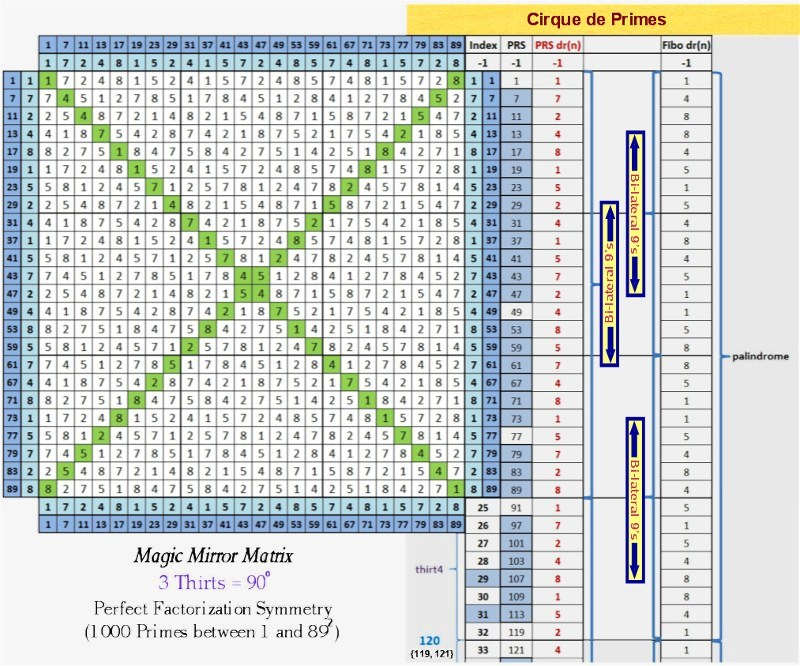### Digital Root Parsing of the Twin Primes

Parsing Twin Primes by their digital roots (in context with natural numbers not divisible by 2, 3 or 5) reveals extremely interesting patterns. Study the matrix below, and you'll discover that it's a beautiful construct of perfectly balanced, multi-directional, 'logic tight' symmetries:Note 1: As indicated, the pink squares marked with x's denote elements with terminating digits = td(5) (their values neither shown nor added into the row sums, above). Although they are not members of our defined set, they are nonetheless interesting. For one thing, the first six td(5) elements sum to 360, as follows: 25 + 35 + 55 + 65 + 85 + 95 = 360. For another, they are always adjacent to modulo 30 "Isolated Primes," discussed below.

Note 2: The first six Isolated Primes, starting with 23, also compile to 360, as follows: 23 + 37 + 53 + 67 + 83 + 97 = 360.

Note 3: Seven is the only number arrayed along the two Isolated Prime radii that isn't 'isolated,' given it is twinned with number 5. Were we to include 7 in our summing of isolated primes, above, the result would be 367, which is itself prime and the sum of seven prime numbers.

Note 4: The first 12 elements that are not td(5), consisting of 11 consecutive prime numbers plus 72, also sum to 360, as follows: 11 + 13 + 17 + 19 + 23 + 29 + 31 + 37 + 41 + 43 + 47 + 49 = 360.

Note 5: Every row possesses "sum symmetry" involving three identical sums. The first row, for example, gives us three sums (working outside-in) totaling 36: 11 + 25; 13 + 23; and 17 + 19 = 36 (and given 36 is 360/10, it comes as no surprise that the 10th row has three sums totaling 360). The 2nd row has three sums totaling 72; the 3rd row has three sums totaling 108, etc. Each successive row thus increments +36, and the row sums accumulate in overlapping cycles of 360 and 432 (the curious relationship between these two numbers discussed elsewhere on this site).

### The 900 Block

When we described the Digital Root Parsing Matrix above as 'logic tight,' we were not overstating it. This is evidenced by the fact that, when elements with a terminating digit of 5 are included, every element in the first 49 rows of the matrix can be coupled with (added to) another element in the matrix to total 900 (with the paired elements spatially located in "equal and opposite" positions). Obviously, each pair totaling 900 must be comprised of elements producing digital root sums that decompose to dr(9). We've dubbed the 48 dyads consisting of two prime numbers totaling 900 in this block '900 Block Primes' (listed below).

[Note: Please keep in mind that it is completely arbitrary of us to fucus on the '900 Block' given there are an infinite number of blocks in an infinite number of incremented configurations within the bounds of the infinitely expandable matrix (e.g., from 47 in Row 3 to 313 in Row 17 forms a '360 Block' and 11 in Row 1 to 1789 in Row 99 (not shown above) would create an '1800 Block').]The Digital Root Parsing Matrix is structured such that any rectangular configuration with 'n' number of columns and 'n' number of rows will produce lateral dyadic sum symmetry, determined by the three dr(9) sum variations: {2,7}, {4,5} and {8,1} (Note: To calculate the bilateral sums for a row, multiply the row's index number times 36.). Here are two examples involving complete (6-element) rows: Take the 17 rows from 2 thru 18 and you'll produce 51 combinations summing to 360; or take the 5 rows from 19 thru 23, which produces 15 combinations summing to 756 (To calculate the number of combinations for any and all rectangles configured from complete rows, multiply the given number of rows times 3.). Now lets carve out a rectangle that doesn't utilize complete rows: we create a 4-column/6-row rectangle with the four corners = 11, 19, 101 and 109 (all prime), and find that all cross-lateral sums equal 120.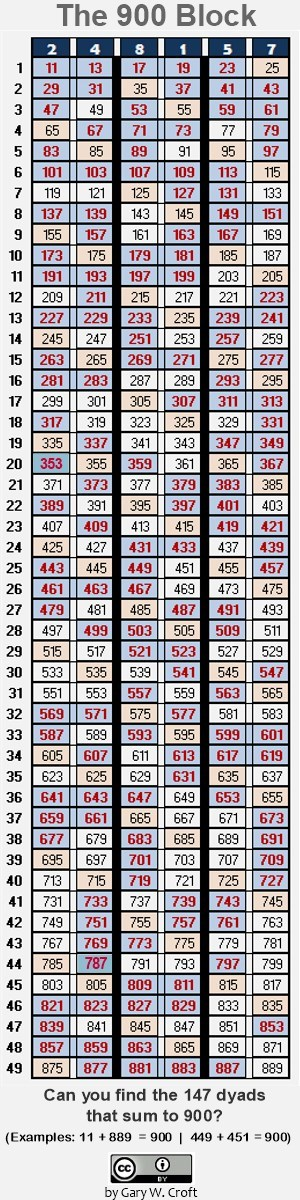What does this object tell us about twin primes? First, we see that twin primes fall into three categories when parsed by their digital roots: {2,4}, {8,1} and {5,7} (2x4, 8x1 and 5x7 all equal dr(8), while 2+4=dr(6); 8+1=dr(9) and 5+7=dr(3): the three legs of the {3,6,9} triangulation which is a member of the Trinity of Triangles, discussed toward the bottom of this page. Next we learn that every twin prime candidate set wihin the '900 Block' has a twin prime candidate pair 'in opposition' creating two sums of 900. Of those falling into this category within the block above, our favorite consists of four sets of twin primes, thus:### Parsing Digital Root Factorization Dyads

As we demonstrated above, employing the logic of digit sum arithmetic, there are a finite number of factorization combinations that correlate to each of the six digital roots, for a total of 6 x 6 = 36 dyads.

The diagram below shows the first 26 twin prime candidates (13 pairs) from the Digital Root Parsing Matrix including the 6 composite numbers falling within this range (from {11,13} to {131,133}). For each composite number we show both its digital root and natural factors.Examining the six digital root dyad factorization groups shown above, we discover that the six sets of multiplicands in every case consist of the triangles {1,4,7} and {2,5,8} (and their rotating variations) interlineated, as we show below ({1,4,7} triangles are in bold to help delineate them):

Digital Root Product = 2: {2, 1, 5, 4, 8, 7}

Digital Root Product = 4: {4, 2, 1, 8, 7, 5}

Digital Root Product = 8: {8, 4, 2, 7, 5, 1}

Digital Root Product = 1: {1, 5, 7, 2, 4, 8}

Digital Root Product = 5: {5, 7, 8, 1, 2, 4}

Digital Root Product = 7: {7, 8, 4, 5, 1, 2}

When the matrix of digital root products is interpreted as a numerical block, we see that all rows and columns sum to 27, and working from the outside in (both vertically and horizontally) we find perfect symmetry involving bilateral 9 sums. This digital root factorization object displays the six possible rotational variations for both {1,4,7} and {2,5,8} triangulations and–speaking of symmetry–does so palindromically. Thus:

{1,4,7}, {4,1,7}, {4,7,1}, {1,7,4}, {7,1,4}, {7,4,1} ... ← palindrome

{2,5,8}, {2,8,5}, {8,2,5}, {5,2,8}, {5,8,2}, {8,5,2} ... ← palindrome

Stringing together the six digital root multiplicand sets produces this beautiful 36-digit palindrome:

215487421875842751157248578124784512.

The significance of these triangles, as members of rotational symmetry groups that can be configured to create a triad of magic squares, is discussed at length toward the bottom of this page.

As we've discussed previously, every pair of twin prime candidates has one element each from the {1,4,7} and {2,5,8} alternating triangulations (and as indicated, all squares distribute to {1,4,7}–never to {2,5,8}).

Most notably, perhaps, digital root parsing reveals the clustering of twin prime candidates into "six packs," i.e., groupings into three prospective prime pairs demarcated by the isolated prime radii. [Note: The only twin prime set in our domain not in a six-pack {17, 19} sums to 36, which, as 1/10th of 360, seems poetic. If we were to decrement {17, 19} in two -18 steps, the result would be 17 + 19 - 1 + 1 - 17 - 19 = 0, which makes logical sense (from zero to 180 being consistent with the set incrementation, shown below).] The first eleven six packs follow (and you'll find the first three of these in the graphic above). And as we alluded, the sets sequence in +180 increments, thus:

11+13 + 29+31 + 47+49 = 180
41+43 + 59+61 + 77+79 = 360
71+73 + 89+91 + 107+109 = 540
101+103 + 119+121 + 137+139 = 720
131+133 + 149+151 + 167+169 = 900
161+163 + 179+181 + 197+199 = 1080
191+193 + 209+211 + 227+229 = 1260
221+223 + 239+241 + 257+259 = 1440
251+253 + 269+271 + 287+289 = 1620
281+283 + 299+301 + 317+319 = 1800
311+313 + 329+331 + 347+349 = 1980

Framing the above as an 11-row/6-column matrix (excluding the row totals), reveals that its 66 elements form 33 dyads that sum to 360 (e.g, 11+349 = 360). Each column stair-steps in increments of 30 and each row, working from the outside in, has three identical sums (e.g., the first row has three dyads that sum to 60 each). Also, we find that the repeating digital root sequences reflect the Trinity of Triangles {3,6,9} {1,4,7} {2,5,8}:

2+4+2+4+2+4: dr(2) + dr(4) = dr(6)
5+7+5+7+5+7: dr(5) + dr(7) = dr(3)
8+1+8+1+8+1: dr(8) + dr(1) = dr(9).

Pointing our discussion of twin prime candidate sequencing triangles back to the Prime Spiral Sieve, we see that three rotations around the Sieve produce six twin prime candidate-related digital root triangles:

11 (2), 13 (4) ... 17 (8), 19 (1) ... 29 (2), 31 (4)

41 (5), 43 (7) ... 47 (2), 49 (4) ... 59 (5), 61 (7)

71 (8), 73 (1) ... 77 (5), 79 (7) ... 89 (8), 91 (1)

### Twin Prime Domino Theory

Switching metaphors, below we represent our repeating 'six-pack' clusters as dominoes. The first row of dominoes reveals patterns shared by all three of the 3-pair twin prime candidate configurations, namely the terminating digit and modulo 30 values array identically across the three dyads parsed by digital roots. The second row of dominoes reveals the repeating patterns by digital root and then by digital root with the terminating digit values excluded. [Note: Every category below possesses 'equal and opposite' sum symmetry. For example, the modulo 30 configuration has 9 lateral sums of 30 each.]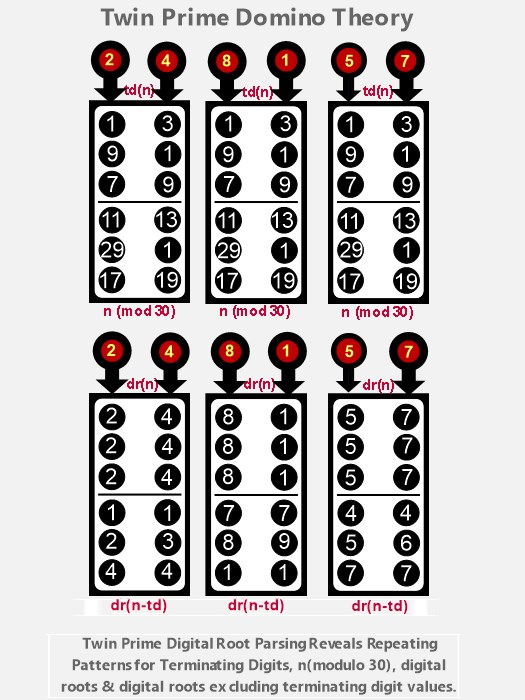It is evident studying the above that the difference sequence between elements in like positions in the 3-pair clusters is 90. We know from digit sum arithmetic that when you increment +90, both the original elements and the +90 incremented sums will possess the same terminating digits, digital roots, n(modulo 30) results, as well as the same digital roots when terminating digit values are excluded. For example, take the twin prime candidate pairs {89, 91} +90 = {179, 181}:

89: dr(8); td(9); 29(mod 30); dr(n-td) = 8
89+90 = 179
179: dr(8); td(9); 29(mod 30); dr(n-td) = 8

91: dr(1); td(1); 1(mod 30); dr(n-td) = 9
91+90 = 181
181: dr(1); td(1); 1(mod 30); dr(n-td) = 9

The dominoes also tell us that:

The lower-valued (left-hand) member of a prime pair will always possess one of three terminating digit ↔ modulo 30 combinations:
td(1) ↔ 11(mod 30)
td(9) ↔ 29(mod 30)
td(7) ↔ 17(mod 30)

The higher-valued (right-hand) member of a prime pair will always possess one of three terminating digit ↔ modulo 30 combinations:
td(3) ↔ 13(mod 30)
td(1) ↔ 1(mod 30)
td(9) ↔ 19(mod 30)

And lastly, twin prime sets that are: n ≡ 29(mod 30) + 2 = n ≡ 1(mod 30) decompose to: dr(n-td) = 2, 3, 5, 6, 8 or 9, while all other twin primes sets [namely n ≡ 11(mod 30) + 2 = n ≡ 13(mod 30) and n ≡ 17(mod 30) + 2 = n ≡ 19(mod 30)] decompose to: dr(n-td) = 1, 4 or 7.

### The Six Digital Root Multiplication Matrices

When we convert the Twin Prime Distribution Channels and/or the paired radii whose angles sum to 360° into digital root multiplication matrices, the results are interesting–to say the least. At the digital root level there are six interrelated matrices of special interest to us: three represent the twin prime distribution channels; the fourth captures the two 'isolated' radii of the Prime Spiral Sieve, i.e. where n ≡ 7 (modulo 30), at 84°, and n ≡ 23 (modulo 30), at 276° (isolated, because, with the exception of 7, which is twinned with 5, the closest proximity they can be to other primes is plus or minus 4, and therefore they can never be twin primes); the fifth and sixth matrices complete the set of four derived from radii whose angles = 360° and in modulo 30 terms sum to 30.

Here's a diagram showing the derivation of the six matrices:

img src="Origin_Digital_Root_Matrices.jpg" height="789" width="475" alt="Diagram showing origin of the six digital root matrices"/>

Each of the six matrices draws 24 elements from the first 96 elements of our domain (starting with 1 and ending with 359; you'll find a deep analysis of these 96 elements under the heading 'Cirque de Primes,' above ...). When matrix multiplied, each sequence produces sixteen identical 36-element squares comprised of six 6-element sequences. The matrix derived from the twin prime distribution channel where n ≡ 1 (modulo 30), at 12°, and n ≡ 29 (modulo 30), at 348°, is pictured directly below. It has sequences identical to those generated when you take a digital root multiplication matrix (aka Vedic Square) and remove all 3's, 6's and 9's (This "Imaginary Square," as we dubbed it, is discussed in more detail under prime factorization).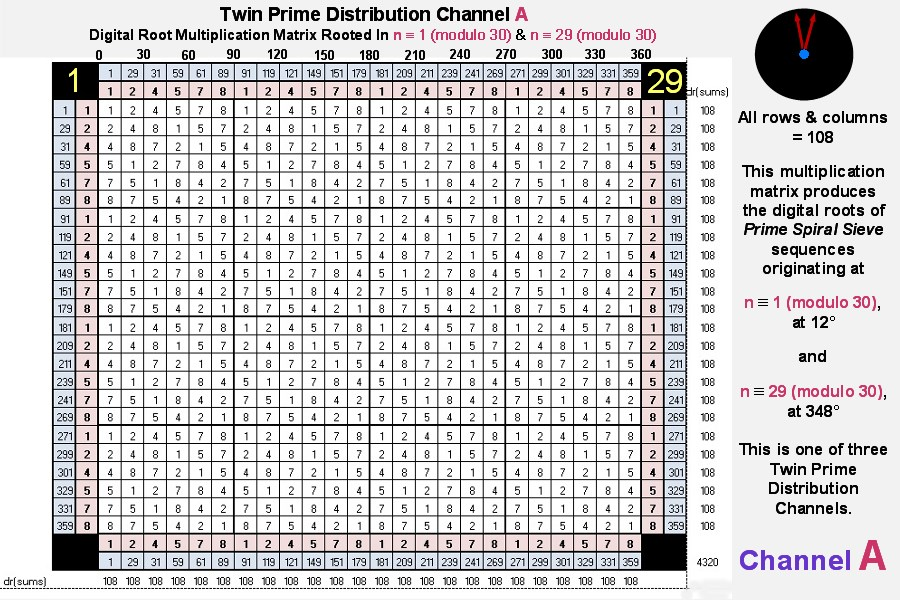The matrices for twin prime distribution channels B & C follow: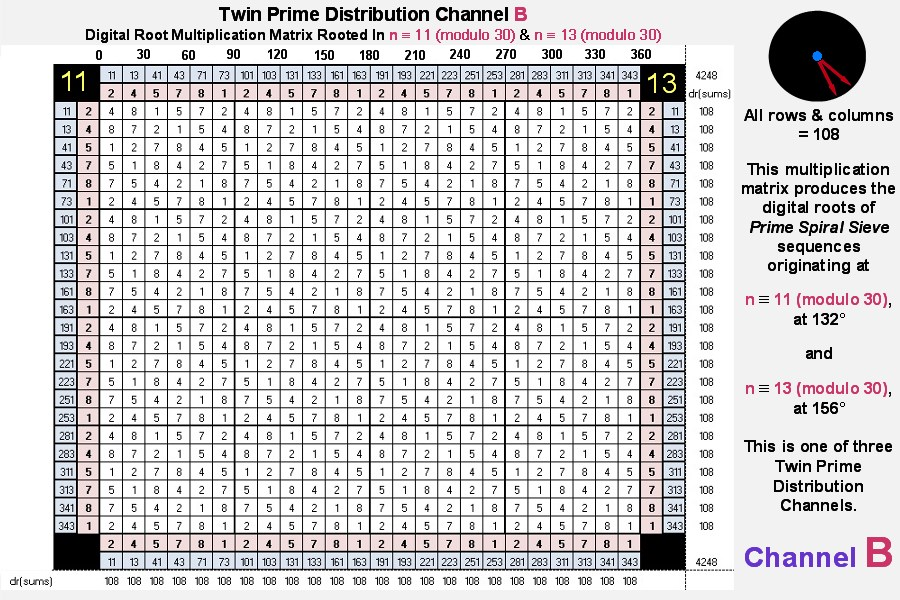Our next matrix is derived from the Isolated Prime Distribution Radii: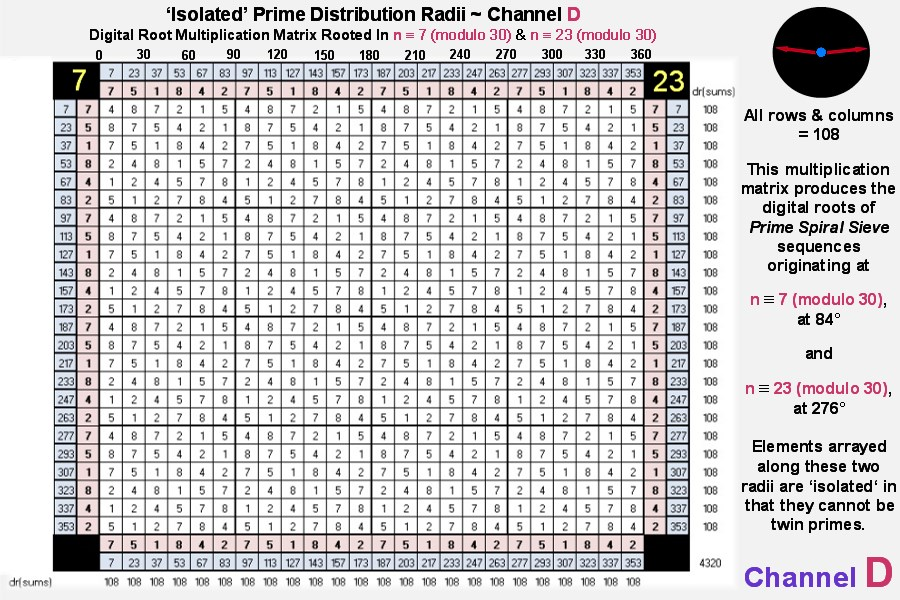And lastly we present the third and fourth matrices derived from paired diagonals whose angles sum to 360°: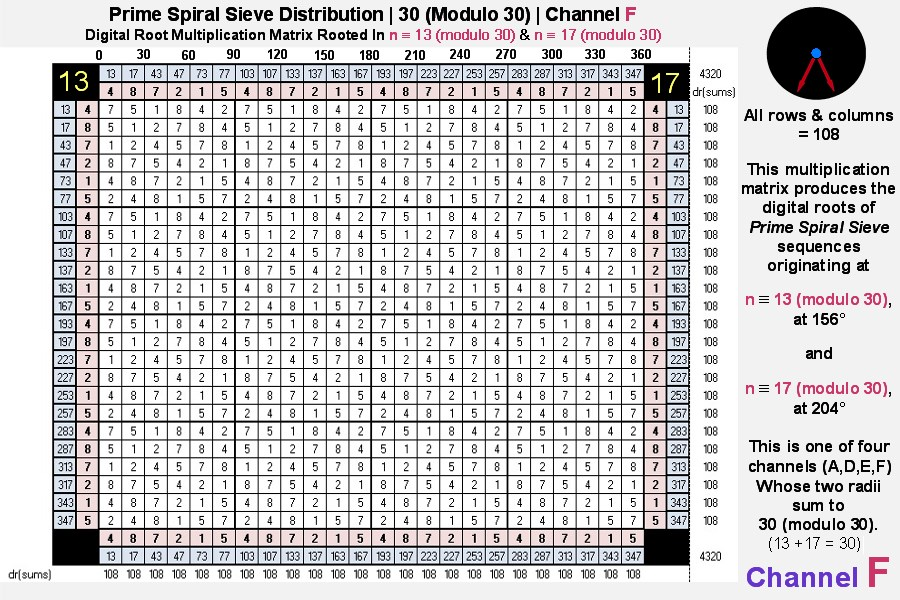Each of the digital root multiplication matrices produced by the six channels consists of what are known in mathematics as 'Orthogonal Latin Squares' (defined in Wikipedia as "an n x n array filled with n different symbols, each occurring exactly once in each row and exactly once in each column" ... in our case every row and column of the repeating 6x6 matrices possesses the six elements: 1, 2, 4, 5, 7, 8 in some order). Also, the sum of the multiplicative digital roots = 108 x 24 = 2592 = 432 x 6.

[Note: Channels A, D, E and F combined represent the set of natural numbers not divisible by 2, 3 and 5, the first 24 elements of which form the basis of the Magic Mirror Matrix.]

The graphic below illustrates the transformative relationships between the matrices employing their primary building blocks (one of the sixteen identical 6 x 6 (36 element) Latin Squares that constitute each matrix):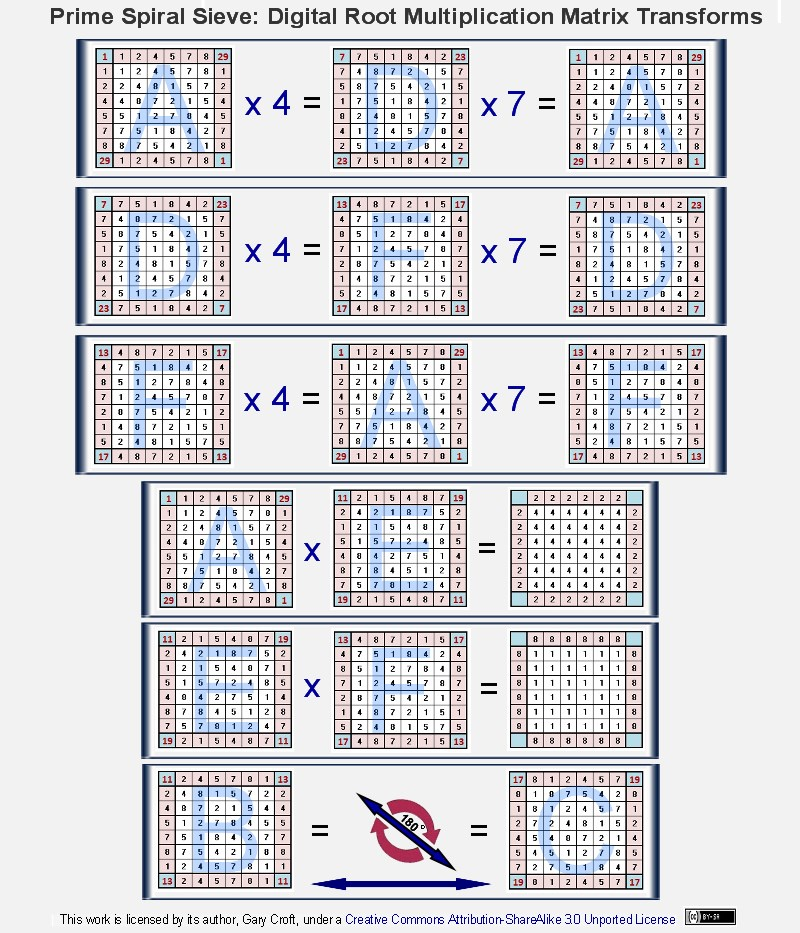Focusing on just the twin prime distribution channels, we see the relationships shown below [and, directly above, we show that two of the channels (B & C) transform bi-directionally by rotating 180° around one of their principal (lower-left to upper-right) diagonal axes]:### Fibonacci Digital Root Multiplication Matrix and Parsing Symmetries

In our discussion under Cirque de Primes, above, we noted that the digital root of Fibonacci numbers indexed by natural numbers not divisible by 2, 3 or 5 form a 32-beat palindromic sequence that repeats every 120° and sums to 432 every 360°. When matrix multiplied this sequence forms a pyramidal mandala of reflectional symmetries that is vertically, horizontally and diagonally palindromic, not to mention each quadrant is palindromic, consisting of perfect symmetry in the form of 8 x 16 x 2 = 256 bilateral 9 sums. Look deeper, and you'll discover yet other vertical and horizontal symmetries. If there were room here, we'd expand this matrix times 3 to encompass 360°. Its 96 palindromic rows and 96 palindromic columns would all equal the magic number 432 ... (We've documented this sequence on the On-Line Encyclopedia of Integer Sequences as A227896.)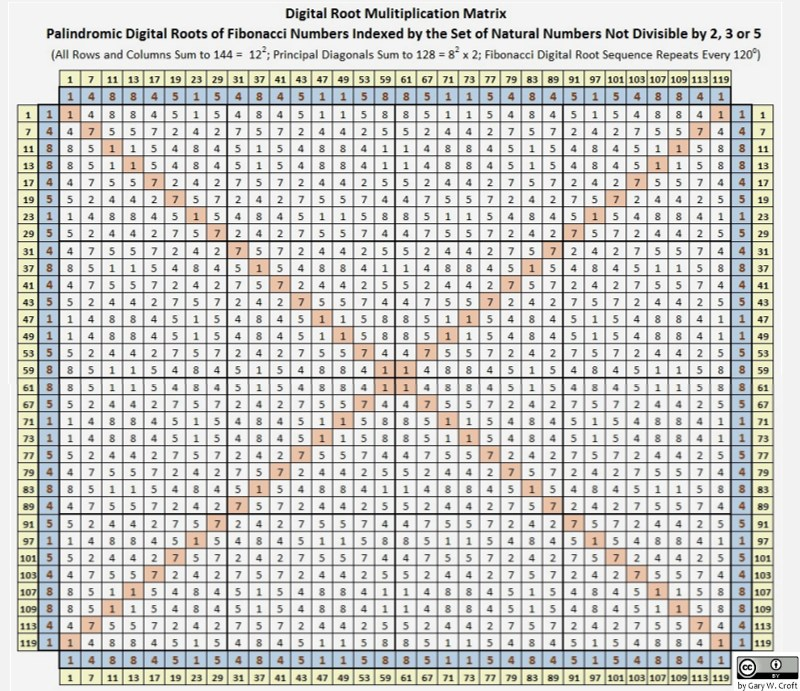When we index the Fibonacci digital roots matrixed above by the twin prime digital root parsing method described earlier, where we parse by digital root dyads {2,4}{1,8} and {5,7}, bilateral sum symmetries are revealed:To complete the picture (the 4th dimension of our 108 x 4 = 432 geometry), we illustrate the Fibonacci digital root sequencing for the 'Isolated Prime' candidates: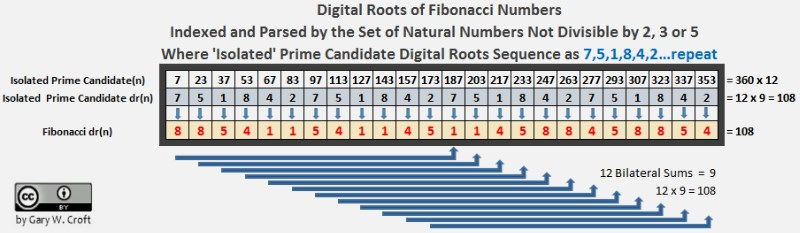### Distribution of Squared Prime Numbers via Fibonacci Digital Roots

Analysis of the modulo 120 sequences discussed above reveals that the digital root of Fibonacci numbers indexed by squared members of natural numbers not divisible by 2, 3 or 5 is always 1, while the squared numbers they're indexed by are always congruent to either 1 (mod 120) or 49 (mod 120), i.e., The squares of all prime numbers >5 are congruent to {1 or 49} mod 120, as follows (shown in relation to modulo 30):

Squares of n ≡ {1,11,19,29} mod 30 are ≡ {1} mod 120
Squares of n ≡ {7,13,17,23} mod 30 are ≡ {49} mod 120

All prime numbers >5 fall into one of the above two categories. (The author has documented this sequence on the On-Line Encyclopedia of Integer Sequences [Sequence #A227863], where it's named: Numbers congruent to {1, 49} mod 120.). [We can also demonstrate that perfect squares of numbers not divisible by 2, 3 or 5 possess a digital root of 1, 4 or 7 and are congruent to either {1} or {19} modulo 30. More on this at Distribution of Perfect Squares.]

This sequence is mysteriously prismatic, as prime numbers embedded in squares are split off in two angles (determined by the terminating digits of their factors), i.e., within a modulo 120 factorization wheel where 1 = 3°, the squares distribute to 3° and 147°, and are thus separated by 144° (And we have to ask whether it's coincidental that the digital roots of the Fibonacci numbers that form the 32-beat repeating palindromic sequence also sum to 144, not to mention, as we've already noted above, when the first three twin prime candidate pairs are summed and multiplied together, 24 x 36 x 60 = 51840, and 51840/360 = 144. This last fact will take on even greater significance when we diagram the 'square roots of squares,' second image, below. One more riff on 144: it's the 12th Fibonacci number following 89 and the only Fibonacci number that's a square other than Fibo1. When you divide 144 by 89, a number with magical properties we explore under 'Fibonacci Twins,' below, you produce an approximation of the golden ratio, or phi, with a difference of only +0.000056460660007.). Another fascinating feature of this array is that any even number of–not necessarily contiguous–factors drawn from any one of the 32 angles in this modulo 120 configuration distribute products to 1(mod 120) or 49 (mod 120), along with the squares. Two examples: 1) at 33°: 11 x 131 x 251 x 371 = 134187361, which is congruent to 1 (mod 120) ; ... 2) at 291°: 97 x 217 = 21049, which is congruent to 49 (mod 120). The matrix below illustrates these relationships:Referencing the matrix above, the illustration below sieves the squares congruent to {1,49} mod 120 and takes their square roots. Twin prime candidates are emphasized to show their repeating zig-zag sequencing on the rungs of a perfectly counter-balanced lattice structure mysteriously populated by the set of natural numbers not divisible by 2, 3 or 5: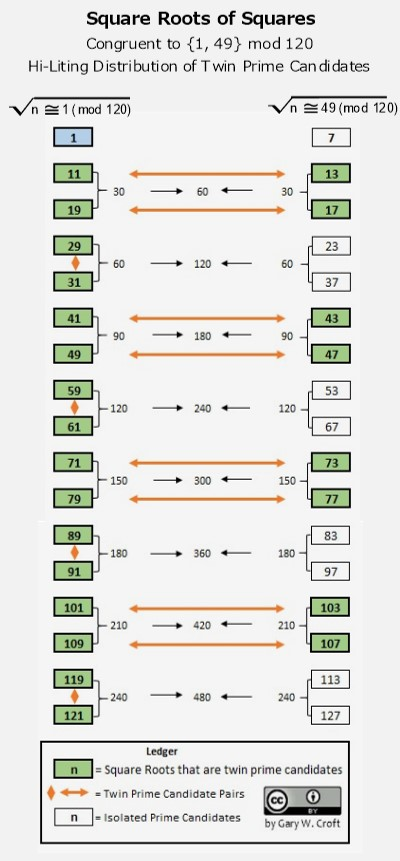### Fibonacci Convergence

It is well known that the ratio of any Fibo(n+2)/Fibo(n) will converge to a limit φ + 1 = φ2 (an irrational number) as n approaches infinity (φ being the symbol for phi or the "golden ratio" which = 1.6180339887498948482045868343656 ...). And so it follows that the ratios of the Fibonacci numbers indexed to the twin primes (n and n+2) and/or twin prime candidates in sequence converge accordingly (in other words, the square roots of the ratios of Fibonacci numbers indexed to the twin primes and/or twin prime candidates in sequence converge to φ).

Here are some examples (square roots not taken):

Fibo(13)/Fibo(11) = 2.617977528089887640449438202247 ...
Fibo(19)/Fibo(17) = 2.618033813400125234815278647464 ...
Fibo(31)/Fibo(29) = 2.618033988748203621343798191078 ...
Fibo(43)/Fibo(41) = 2.618033988749894831892914017992 ...
Fibo(49)/Fibo(47) = 2.618033988749894848153928976786 ...
Fibo(61)/Fibo(59) = 2.618033988749894848204586345776 ...

Here's an interesting connection to Fibo(13)/Fibo(11) = 2.617977528089887640449438202247: Fibo(13) = 233, while Fibo(11) = 89. The square root of Fibo(13) divided by Fibo(11) = 122 divided by 89 (and 89 has the basis of the Fibonacci sequence in the decimal expansion of its reciprocal). Then consider that 3 x 122 = 432, a fascinating number we discuss above under 'Cirque de Primes,' below under 'Fibonacci Twins,' as well as on our page detailing the Magic Mirror Matrix where we also examine the amazing properties of number 89.

### Perfect Twins

One other twin prime related deterministic algorithm worth noting stair-steps its way up the twin prime distribution channels with a periodicity of 6. We've dubbed these "perfect twins," given that the square root of their 'perfect' square sums is the first perfect number, 6, or one of its multiples. To calculate this interesting twin pair candidate sequence, start with x = 6 (then add 6 and repeat for each successive step, as shown below):x=6: 62 = 36; 36/2 = 18; 18-1 = 17; 18+1 = 19; thus, twin pair candidates = 17 and 19; take the square root of 17+19 which = 6

x=12: 122 = 144; 144/2 = 72; 72-1 = 71; 72+1 = 73; thus, twin pair candidates = 71 and 73; take the square root of 71+73 which = 12

add 6 (12+6 = 18) {repeat ... n}

The table below shows the first 20 steps in the sequence. All "perfect twins" are hi-lited in gold. The table is arrayed in five columns to show the +30 and repeating modulo 30 vertical incrementation. Also note that the digital roots for all prime pair candidates and/or prime pairs in this sequence equate to 8 + 1 = 9, e.g., 17, or dr(8) + 19, or dr(1) = 36 or dr(9).[Note: We've documented these "perfect twins" on the On-Line Encyclopedia of Integer Sequences (Sequence #A232878) where they're defined as Twin prime pairs which sum to perfect squares.]

### Infinite Twins

Referencing the Twin Prime Conjecture: Given that all composite numbers extant in the infinite set of natural numbers not divisible by 2, 3 or 5 (which by definition contains all prime numbers ≥ 7 and their multiplicative multiples and is defined as n ≡ {1, 7, 11, 13, 17, 19, 23, 29} modulo 30 and sequences as 1 {+6 +4 +2 +4 +2 +4 +6 +2} {repeat ... ∞}), can be identified algorithmically, employing eight geometrically-expanding factorization sequences, ultimately leaving the equal distribution of all prime numbers ≥ 7 ... n along eight diagonals (and we note here that, regardless, according to a theorem due to Dirichlet any arithmetic progression with no common factor throughout contains infinitely many primes); and given that six of these diagonals with an infinity of primes form the three twin prime distribution channels (described above), which, when combined into a single number line, account for all twin primes > (5,7). This sequence is defined as n ≡ {1, 11, 13, 17, 19, 29} modulo 30 and has a repetition cycle of 1 {+10 +2 +4 +2 +10 +2} {repeat ... ∞} where every 6 beats produces 3 pairs of n, n+2 twin prime candidates.

Given these facts, it would seem axiomatic that there are infinite prime pairs in the form p, p + 2. (Cautionary Note: We are not sufficiently delusional to consider this a mathematical proof. Rather, this statement is an argument for the high probability that the number of twin primes is unnumberable, i.e., infinite.)

### Seeing Stars

Another interesting aspect of the three twin prime distribution channels becomes apparent when the eight radials of the Prime Spiral Sieve (or modulo 30 wheel factorization), are superimposed upon a {10/3} regular star polygon (see illustration, below). Thirty (30) is the total unit length of the ten straight line segments used to construct the {10/3} star polygon, where the straight lines connect every 3rd point of 10 equally spaced points lying on a circle's circumference. Note that three points of the polygon, 144°(12), 216°(18) and 360°(30), precisely and symmetrically split the twin prime distribution channels. And as you rotate this object, several other symmetries become apparent, e.g.: between 7 → 17; 13 → 23; and 7 → 23.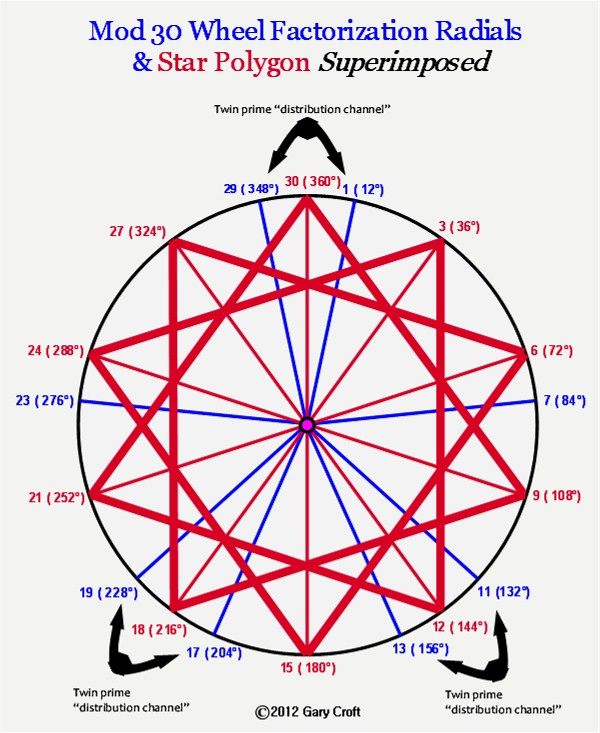Even more interesting, is the result when you superimpose a 15-point star over a Prime Spiral Sieve with its 'aperture' increased to modulo 90. As shown below, every one of the 9 twin prime distribution channels is hit dead center by points of the star:This superimposition reveals some interesting circularities: When you sum the 9 angles that hit the twin prime distribution channels dead center you get 1800. 1800/360=5; 1800/9=200. In turn, sum the 6 angles that hit equidistant between the twin prime distribution channels and you get 1080. 1080/360=3. 1080/6=180. In toto, 1800+1080=2880; 2880/360=8.

### Twin Prime Magic Squares

Before leaving the twin primes, let's take one more look at them from a digital root perspective. The illustration below shows the infinitely repeating digital root sequences for each of the 8 radii of the spiral sieve, as well the sums of the digital roots (in yellow) between each radius, including the twin prime distribution channels. It's notable that the twin pair digital sum sequences, {3,9,6}, {9,6,3} and {6,3,9} when positioned adjacent to each other in ascending order, create a "magic square" (in that all vertical, horizontal and principal diagonal sums equal 18), as pictured below:The {3,6,9} triangulation is a member of the so-called 'Trinity of Triangles,' along with {1,4,7} and {2,5,8}. Looking at the graphic above, you'll note that each of the three Twin Prime Distribution Channels has one leg that sequences as {1,4,7} and the other as {2,5,8}, albeit the starting digits may vary, viz. {1,4,7} vs. {4,7,1}. When you combine the three legs that sequence as {1,4,7} as well as the three legs that sequence as {2,5,8}, these also form magic squares, as illustrated below. Another magic square hidden in plain sight becomes evident when you examine the sequences you encounter in rotation: {1,4,7} ... {2,5,8} ... {3,6,9} in various sequence combinations. Taking the digits 1 thru 9 these three triangles represent, they can be configured into a magic square representing 'et alia', in this case the famous 'Lo Shu Magic Square':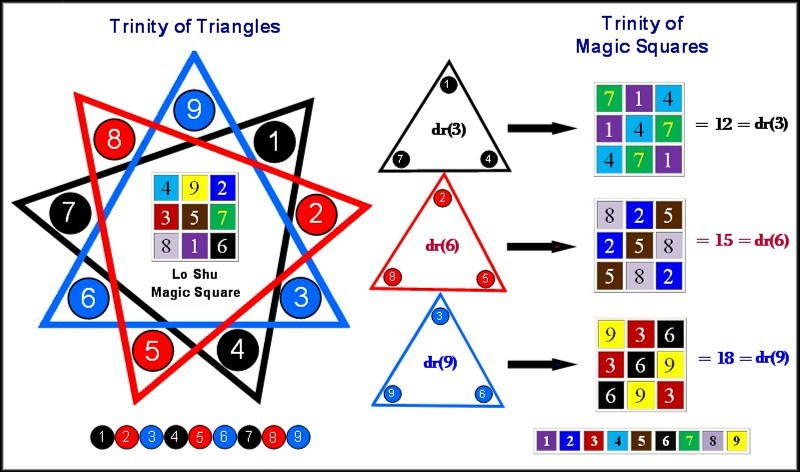Under "Factorization Algorithms for the Twin Prime Candidates," above, we detailed how twin primes come in three digital root configurations: {2,4}, {5,7} and {8,1} and demonstrated that these dyads, in turn, can form the basis of period-24 digital root cycles that generate {9/3} star polygons via 3 x 3 matrices. So with these cycles and matrices in mind, take the three twin prime digital root dyads under discussion, sequence their 24-length digital root cycles, then tier them, and you'll discover a direct means to constructing the Trinity of Magic Squares via the Trinity of Triangles, as illustrated here: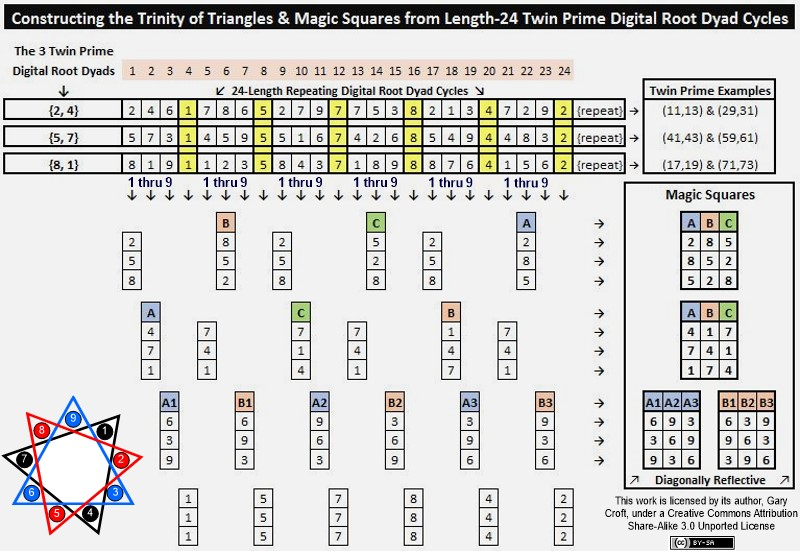When you rotate either the {1,4,7} or {2,5,8} magic square around its horizontal axis, i.e. columns {A,B,C} become {C,B,A}, then add the {1,4,7} {2,5,8} magic squares together, you produce a square with nine 9's. For example, adding the first rows of each gives us: {2,8,5} + {7,1,4} = {9,9,9}.

Triangles and magic squares similar–or identical–to those shown above can be derived from the digital root sequence cycles of all three twin prime distribution channels (namely numbers ≡ to {11,13}, {17,19} and {1,29} modulo 30). This is also true of dyads formed by paired radii of the Prime Spiral Sieve that sum to 30, i.e., numbers ≡ to {1,29}, {7,23}, {11,19}, or {13,17} modulo 30, as well as dyads formed when {n, n + 10} are ≡ to {1, 11}, {7, 17}, {13, 23} or {19, 29} modulo 30 (note their pairing by terminating digits). One example relating to twin primes: The first three candidate pairs in the twin prime distribution channel ≡ to {11,13} modulo 30 (all three of which are indeed twin primes) sequence their digital roots as follows:

{11,13} = digital roots 2 & 4
{41,43} = digital roots 5 & 7
{71,73} = digital roots 8 & 1.

As you can see, this is the same digital root sequence illustrated above.

It appears that the triangulations and magic squares structuring the distribution of twin primes (and as it turns out, all prime numbers) have a genesis in universal principles involving symmetry groups rotated by the 8-dimensional algorithms discussed at length on this site. You can see this universal principle at work, for example, with regard to the Fibonacci digital root sequence when coupled to a pair of dyads that follow certain incremental rules. As we illustrated above, the initializing dyad of the period-24 Fibonacci digital root sequence is {1,1, ...}. We can generate triangles and magic squares by tiering the Fibonacci digital root sequence with two pairs of terms that are + 3 or + 6 from the initial terms {1,1}. The values of the 2nd and 3rd tiers, or rows, must differ, or symmetry is lost. In other words, the first two columns should read either {1,4,7 + 1,7,4, or vice versa} but not {1,4,7 + 1,4,7, or 1,7,4, + 1,7,4}. Here's a picture that displays the {1,4,7 + 1,7,4} digital root sequencing used to construct triangles and magic squares:We'll end our exploration of twin primes with two powerful magic squares. First: The Trinity of Magic Squares x 3 can be constructed into a magic square where all columns, rows and principal diagonals equal 45 (and were you to construct an 8x8 square employing 64 Trinity Magic Squares shown below, all columns, rows and principal diagonals would equal 360).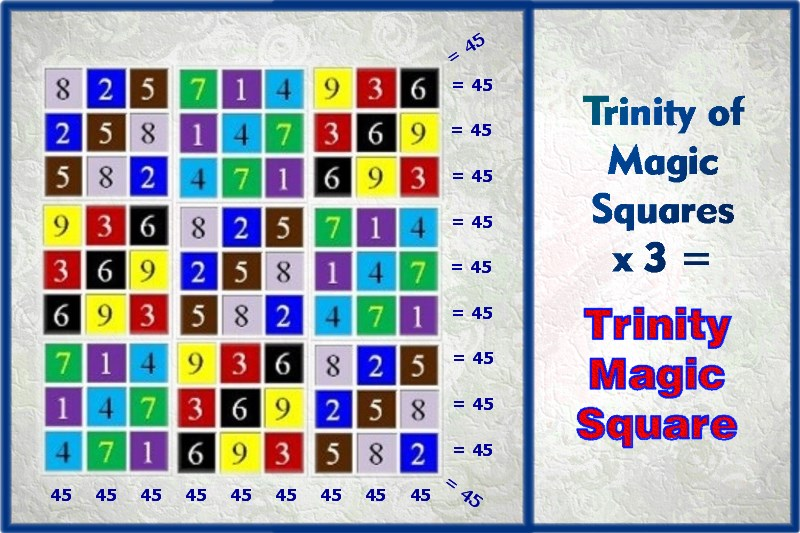Next we show how the Lo Shu Magic Square and Trinity of Magic Squares can be combined in a "4-Fold Way" to create their own magic square when configured in a 4 x 4 matrix of magic squares consisting of 144 elements where all rows, columns and principal diagonals sum to 60 and the sum total of the matrix's elements = 720 = 360 x 2 (and were we to construct a 6x6 square employing 36 4-Fold Way Magic Squares shown below, all columns, rows and principal diagonals would equal 360).